Скачать презентацию Hierarchy of Production Decisions Forecasting First a

20bc49ed259268f12c4c07c862ebe98f.ppt

• Количество слайдов: 74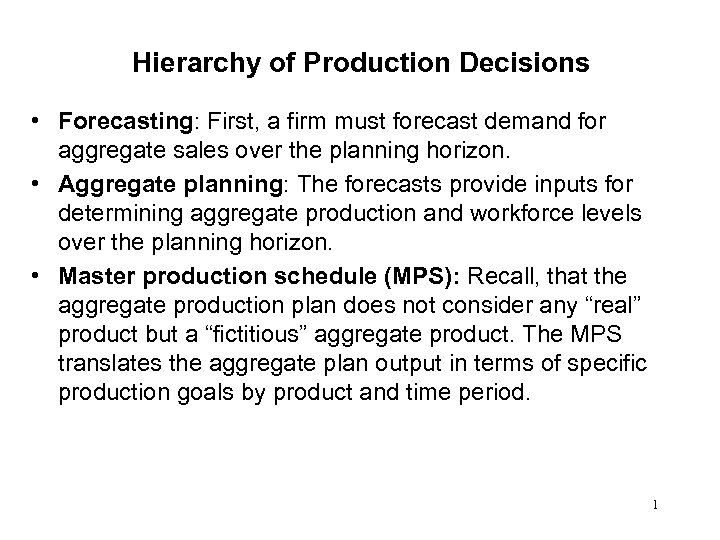Hierarchy of Production Decisions • Forecasting: First, a firm must forecast demand for aggregate sales over the planning horizon. • Aggregate planning: The forecasts provide inputs for determining aggregate production and workforce levels over the planning horizon. • Master production schedule (MPS): Recall, that the aggregate production plan does not consider any “real” product but a “fictitious” aggregate product. The MPS translates the aggregate plan output in terms of specific production goals by product and time period. 1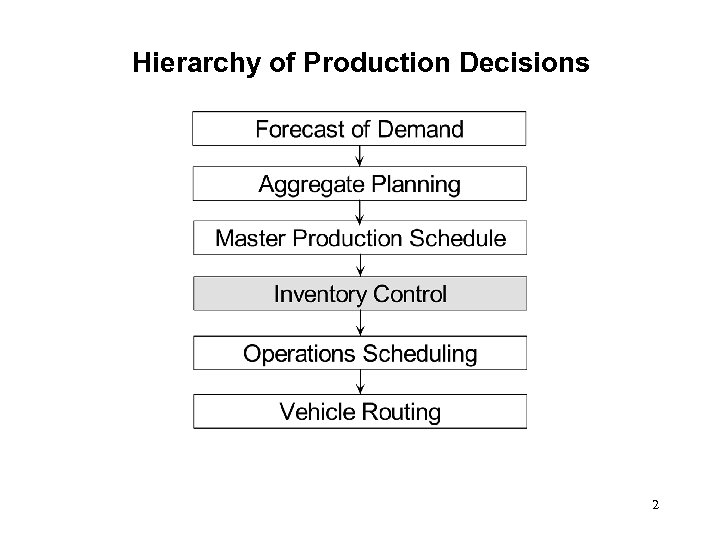Hierarchy of Production Decisions 2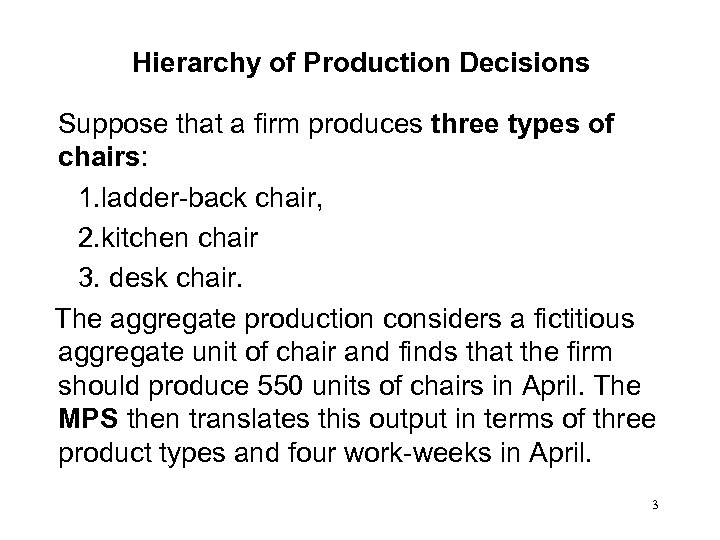Hierarchy of Production Decisions Suppose that a firm produces three types of chairs: 1. ladder-back chair, 2. kitchen chair 3. desk chair. The aggregate production considers a fictitious aggregate unit of chair and finds that the firm should produce 550 units of chairs in April. The MPS then translates this output in terms of three product types and four work-weeks in April. 3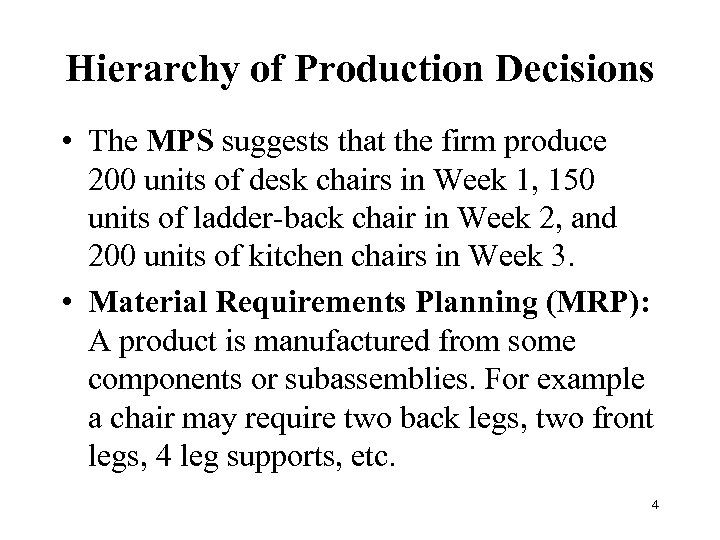Hierarchy of Production Decisions • The MPS suggests that the firm produce 200 units of desk chairs in Week 1, 150 units of ladder-back chair in Week 2, and 200 units of kitchen chairs in Week 3. • Material Requirements Planning (MRP): A product is manufactured from some components or subassemblies. For example a chair may require two back legs, two front legs, 4 leg supports, etc. 4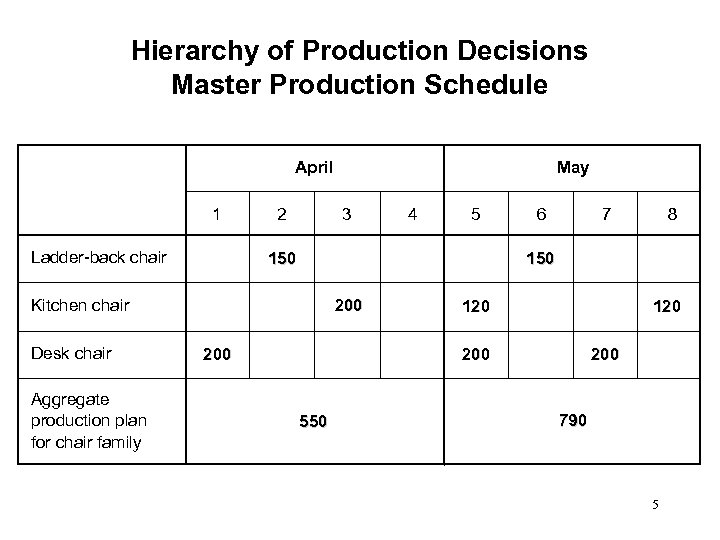Hierarchy of Production Decisions Master Production Schedule April 1 Ladder-back chair 2 3 Aggregate production plan for chair family 4 5 150 6 7 8 150 200 Kitchen chair Desk chair May 200 120 200 550 200 790 5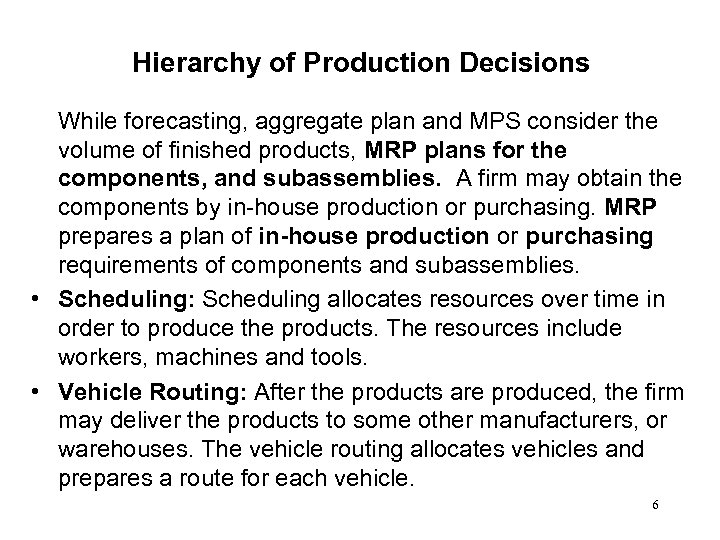Hierarchy of Production Decisions While forecasting, aggregate plan and MPS consider the volume of finished products, MRP plans for the components, and subassemblies. A firm may obtain the components by in-house production or purchasing. MRP prepares a plan of in-house production or purchasing requirements of components and subassemblies. • Scheduling: Scheduling allocates resources over time in order to produce the products. The resources include workers, machines and tools. • Vehicle Routing: After the products are produced, the firm may deliver the products to some other manufacturers, or warehouses. The vehicle routing allocates vehicles and prepares a route for each vehicle. 6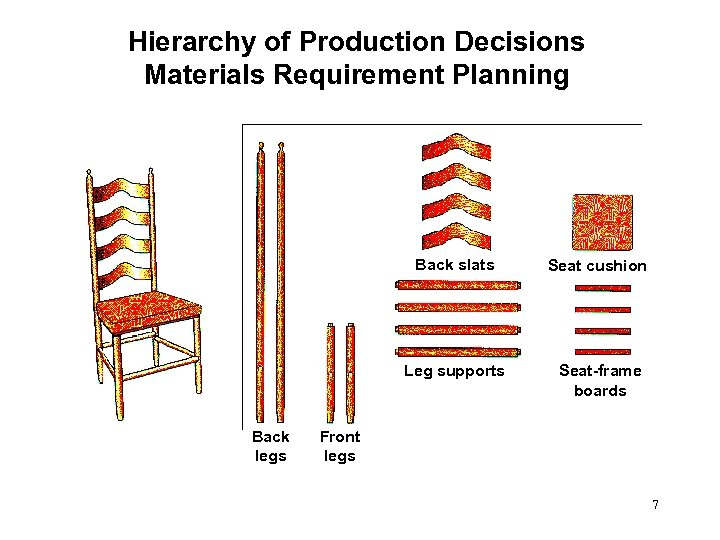Hierarchy of Production Decisions Materials Requirement Planning Back slats Leg supports Back legs Seat cushion Seat-frame boards Front legs 7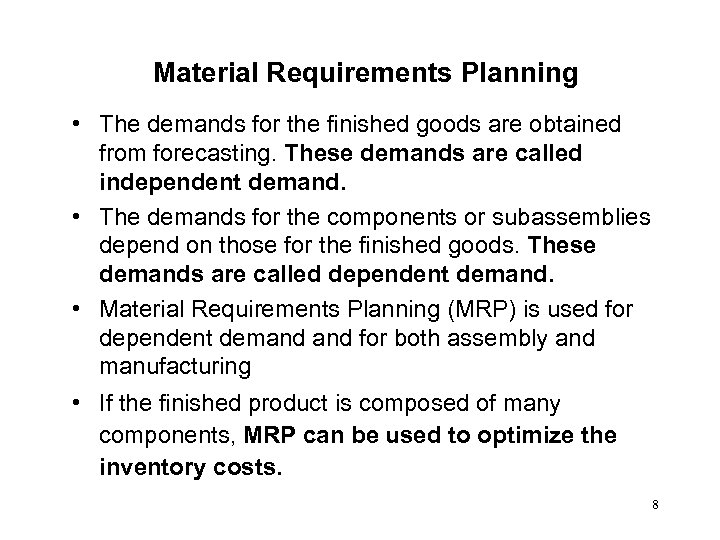Material Requirements Planning • The demands for the finished goods are obtained from forecasting. These demands are called independent demand. • The demands for the components or subassemblies depend on those for the finished goods. These demands are called dependent demand. • Material Requirements Planning (MRP) is used for dependent demand for both assembly and manufacturing • If the finished product is composed of many components, MRP can be used to optimize the inventory costs. 8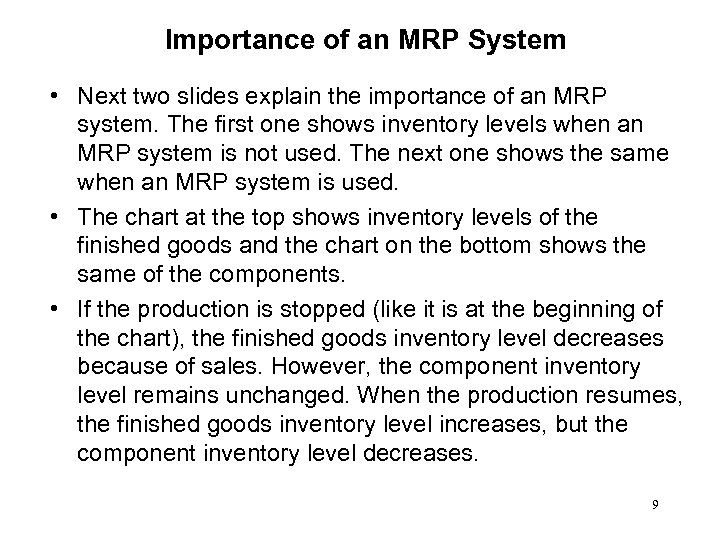Importance of an MRP System • Next two slides explain the importance of an MRP system. The first one shows inventory levels when an MRP system is not used. The next one shows the same when an MRP system is used. • The chart at the top shows inventory levels of the finished goods and the chart on the bottom shows the same of the components. • If the production is stopped (like it is at the beginning of the chart), the finished goods inventory level decreases because of sales. However, the component inventory level remains unchanged. When the production resumes, the finished goods inventory level increases, but the component inventory level decreases. 9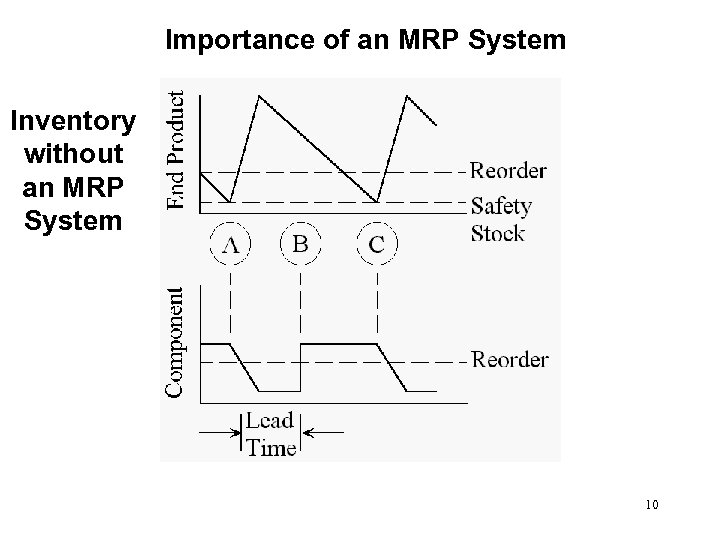Importance of an MRP System Inventory without an MRP System 10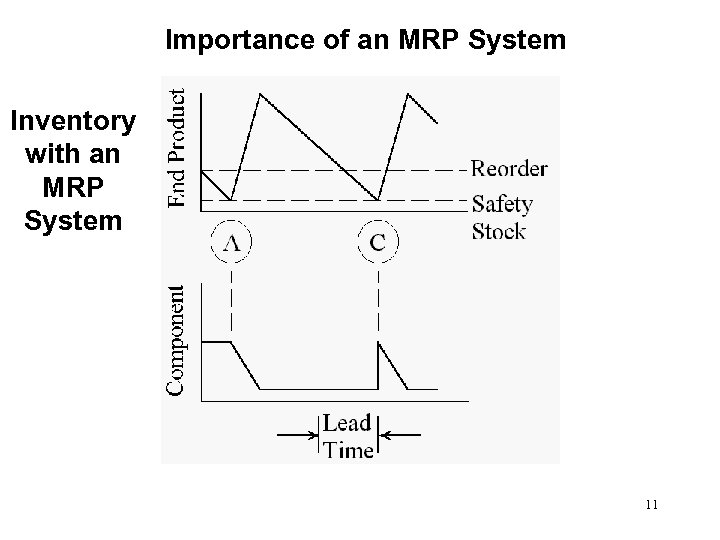Importance of an MRP System Inventory with an MRP System 11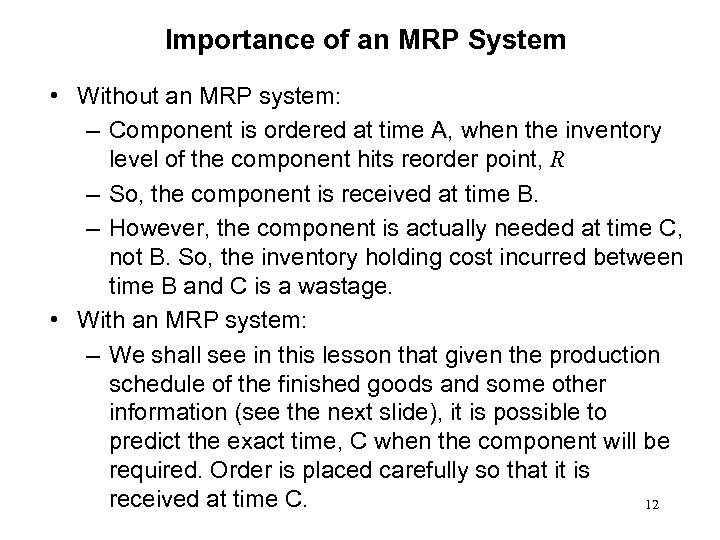Importance of an MRP System • Without an MRP system: – Component is ordered at time A, when the inventory level of the component hits reorder point, R – So, the component is received at time B. – However, the component is actually needed at time C, not B. So, the inventory holding cost incurred between time B and C is a wastage. • With an MRP system: – We shall see in this lesson that given the production schedule of the finished goods and some other information (see the next slide), it is possible to predict the exact time, C when the component will be required. Order is placed carefully so that it is received at time C. 12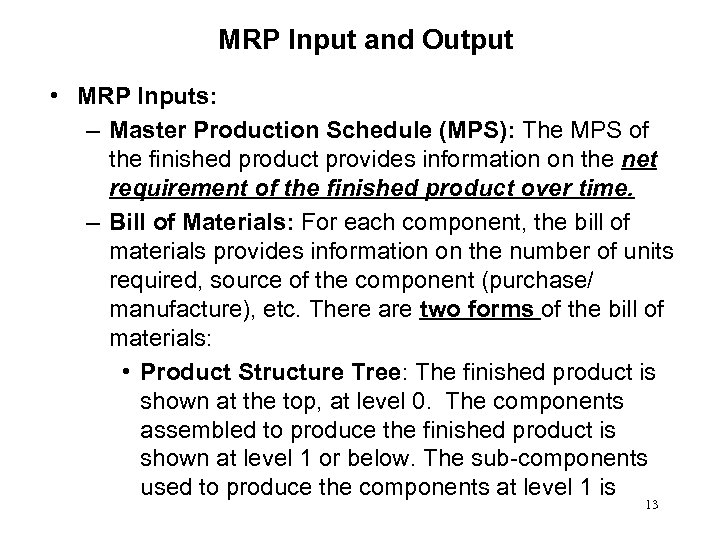MRP Input and Output • MRP Inputs: – Master Production Schedule (MPS): The MPS of the finished product provides information on the net requirement of the finished product over time. – Bill of Materials: For each component, the bill of materials provides information on the number of units required, source of the component (purchase/ manufacture), etc. There are two forms of the bill of materials: • Product Structure Tree: The finished product is shown at the top, at level 0. The components assembled to produce the finished product is shown at level 1 or below. The sub-components used to produce the components at level 1 is 13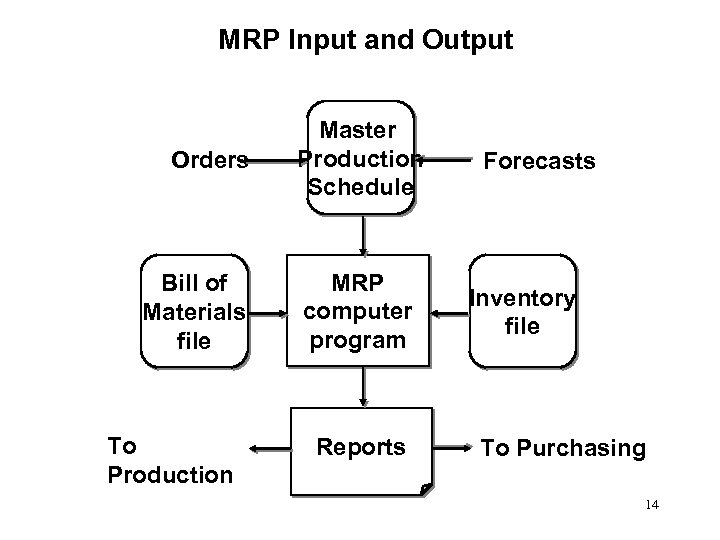MRP Input and Output Orders Bill of Materials file To Production Master Production Schedule MRP computer program Reports Forecasts Inventory file To Purchasing 14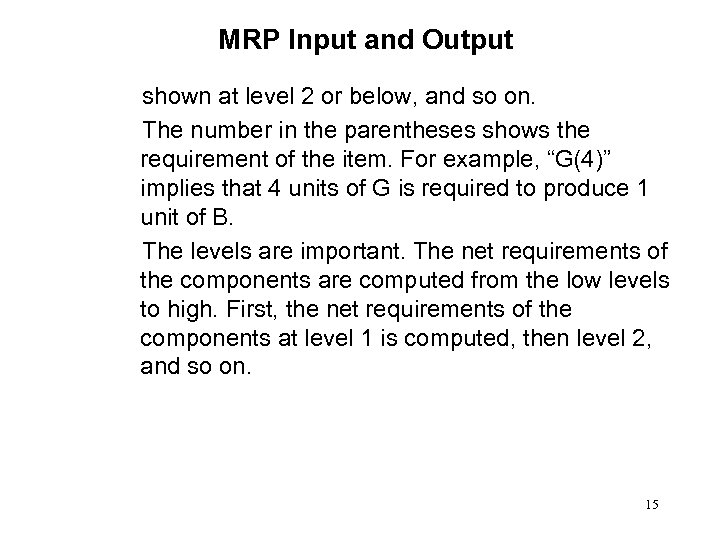MRP Input and Output shown at level 2 or below, and so on. The number in the parentheses shows the requirement of the item. For example, “G(4)” implies that 4 units of G is required to produce 1 unit of B. The levels are important. The net requirements of the components are computed from the low levels to high. First, the net requirements of the components at level 1 is computed, then level 2, and so on. 15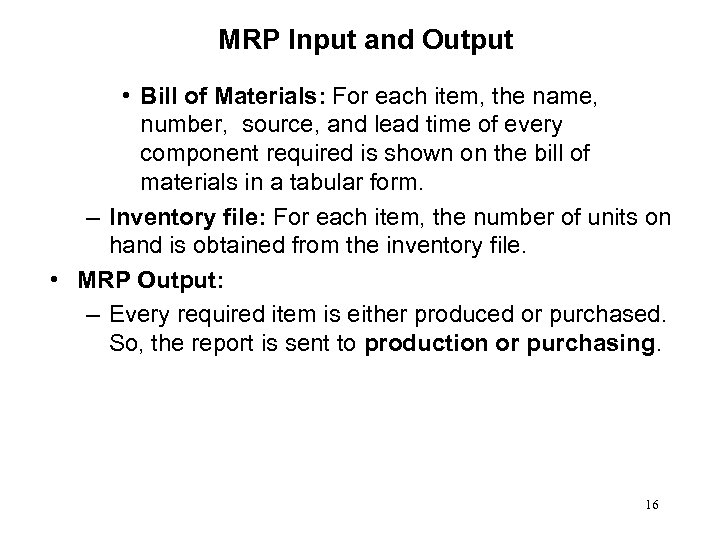MRP Input and Output • Bill of Materials: For each item, the name, number, source, and lead time of every component required is shown on the bill of materials in a tabular form. – Inventory file: For each item, the number of units on hand is obtained from the inventory file. • MRP Output: – Every required item is either produced or purchased. So, the report is sent to production or purchasing. 16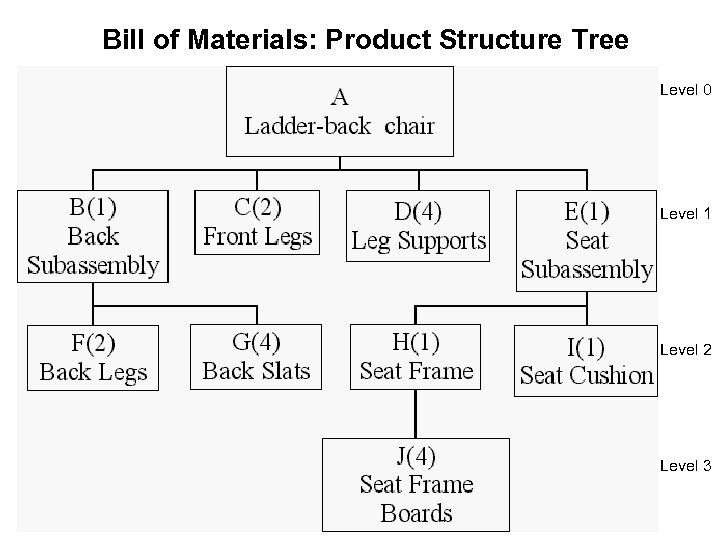Bill of Materials: Product Structure Tree Level 0 Level 1 Level 2 Level 3 17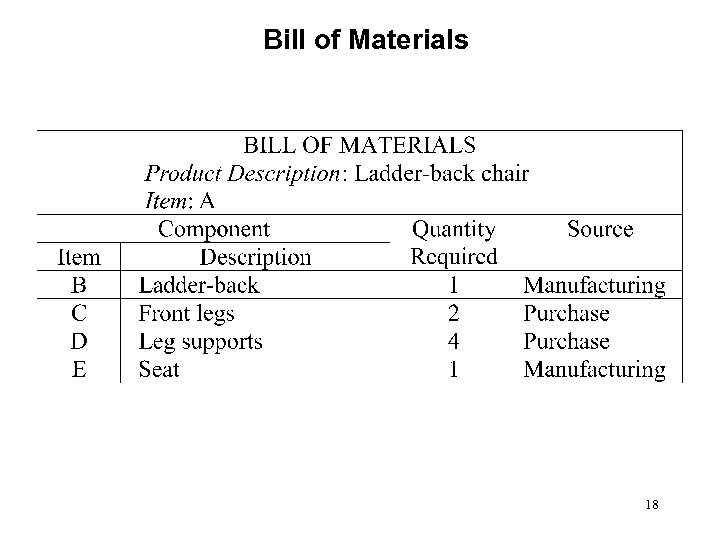Bill of Materials 18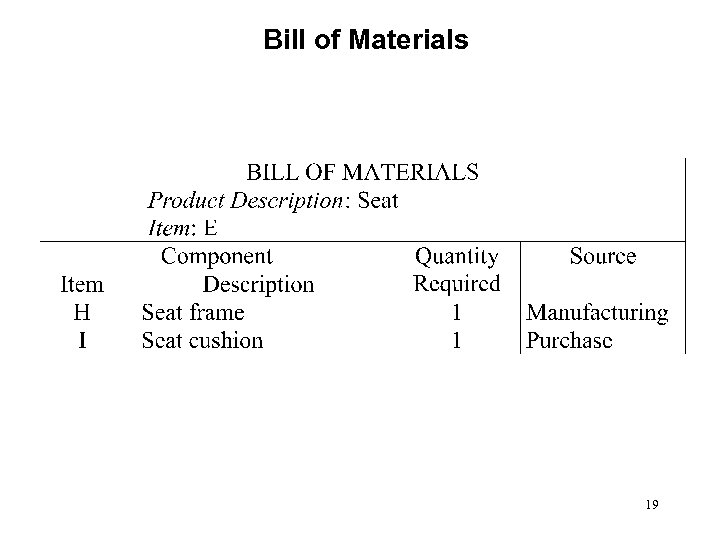Bill of Materials 19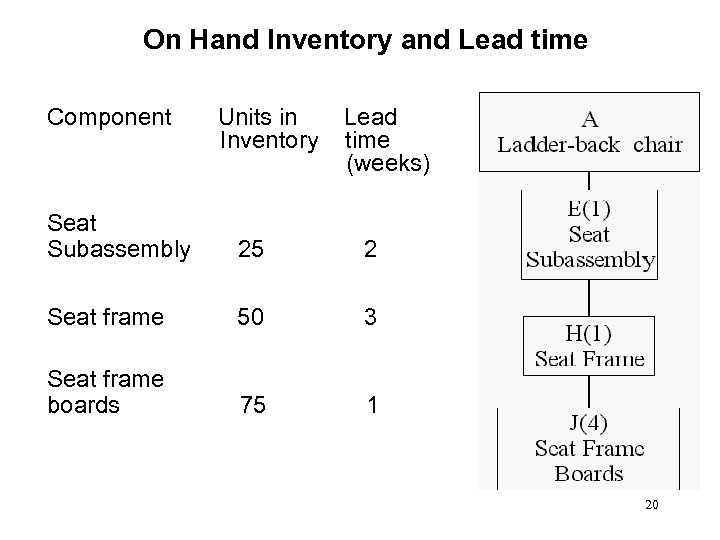On Hand Inventory and Lead time Component Units in Inventory Lead time (weeks) Seat Subassembly 25 2 Seat frame 50 3 Seat frame boards 75 1 20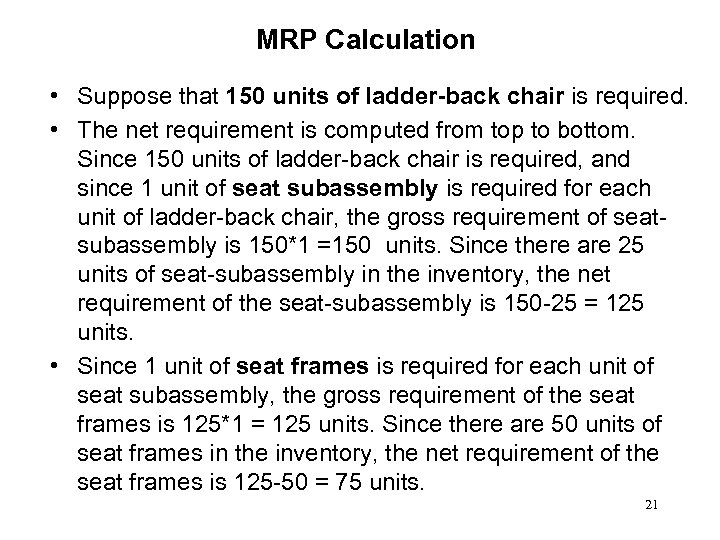MRP Calculation • Suppose that 150 units of ladder-back chair is required. • The net requirement is computed from top to bottom. Since 150 units of ladder-back chair is required, and since 1 unit of seat subassembly is required for each unit of ladder-back chair, the gross requirement of seatsubassembly is 150*1 =150 units. Since there are 25 units of seat-subassembly in the inventory, the net requirement of the seat-subassembly is 150 -25 = 125 units. • Since 1 unit of seat frames is required for each unit of seat subassembly, the gross requirement of the seat frames is 125*1 = 125 units. Since there are 50 units of seat frames in the inventory, the net requirement of the seat frames is 125 -50 = 75 units. 21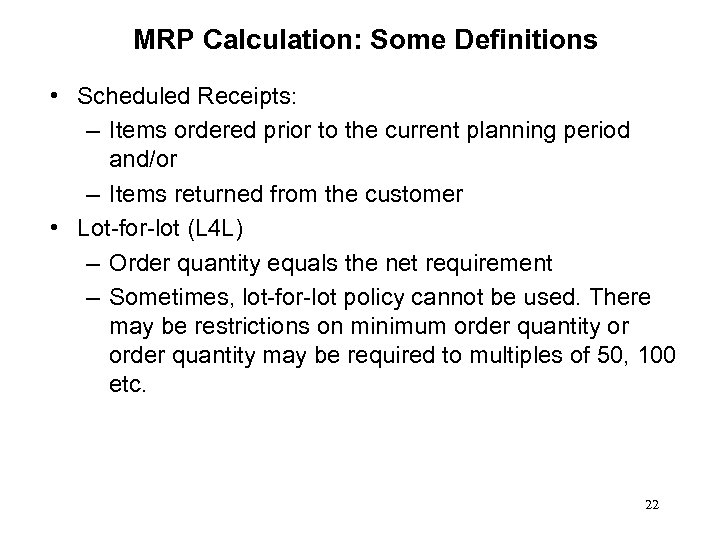MRP Calculation: Some Definitions • Scheduled Receipts: – Items ordered prior to the current planning period and/or – Items returned from the customer • Lot-for-lot (L 4 L) – Order quantity equals the net requirement – Sometimes, lot-for-lot policy cannot be used. There may be restrictions on minimum order quantity or order quantity may be required to multiples of 50, 100 etc. 22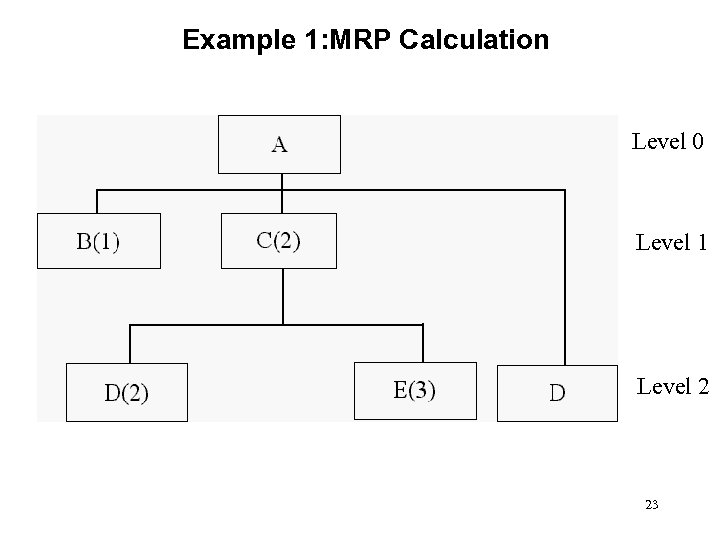Example 1: MRP Calculation Level 0 Level 1 Level 2 23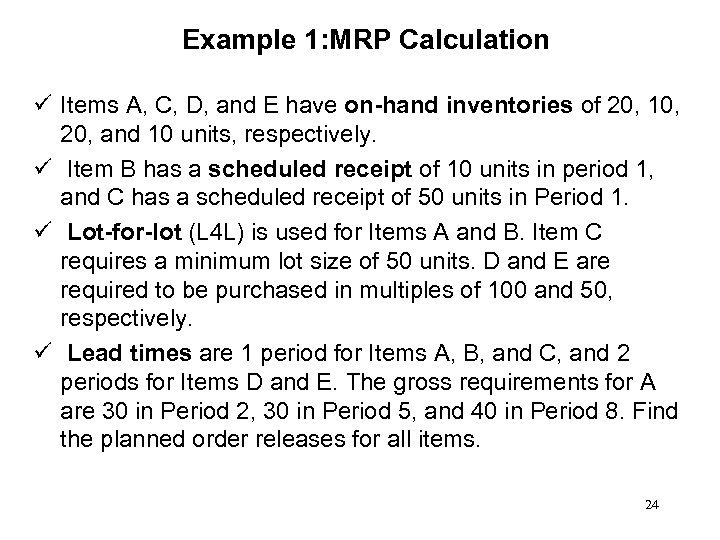Example 1: MRP Calculation ü Items A, C, D, and E have on-hand inventories of 20, 10, 20, and 10 units, respectively. ü Item B has a scheduled receipt of 10 units in period 1, and C has a scheduled receipt of 50 units in Period 1. ü Lot-for-lot (L 4 L) is used for Items A and B. Item C requires a minimum lot size of 50 units. D and E are required to be purchased in multiples of 100 and 50, respectively. ü Lead times are 1 period for Items A, B, and C, and 2 periods for Items D and E. The gross requirements for A are 30 in Period 2, 30 in Period 5, and 40 in Period 8. Find the planned order releases for all items. 24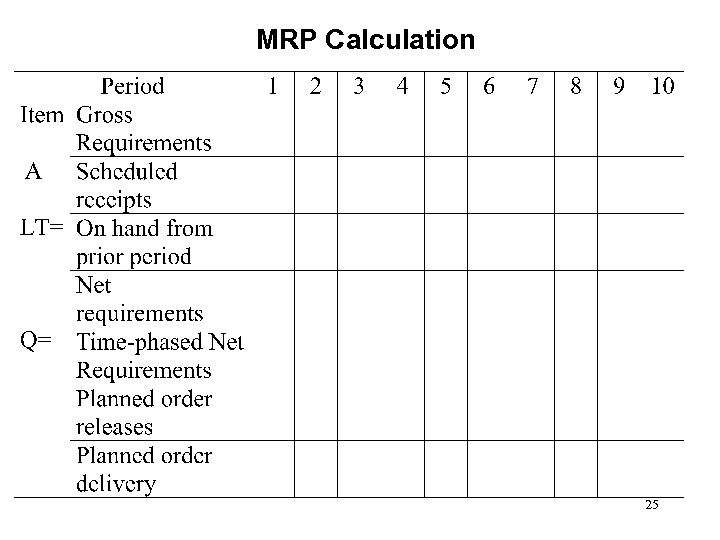MRP Calculation 25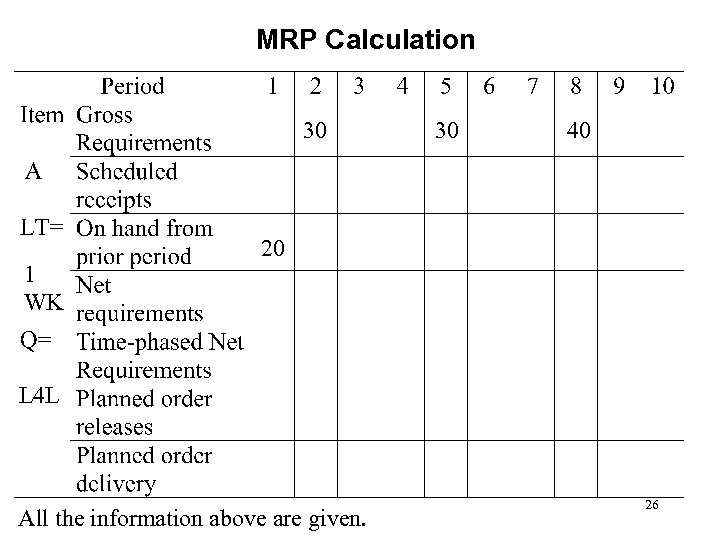MRP Calculation 30 1 WK 30 40 20 L 4 L All the information above are given. 26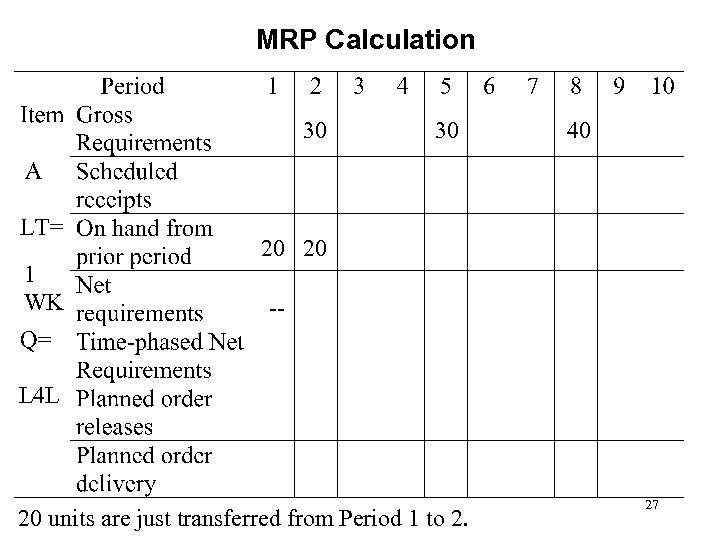MRP Calculation 30 1 WK 30 40 20 20 -- L 4 L 20 units are just transferred from Period 1 to 2. 27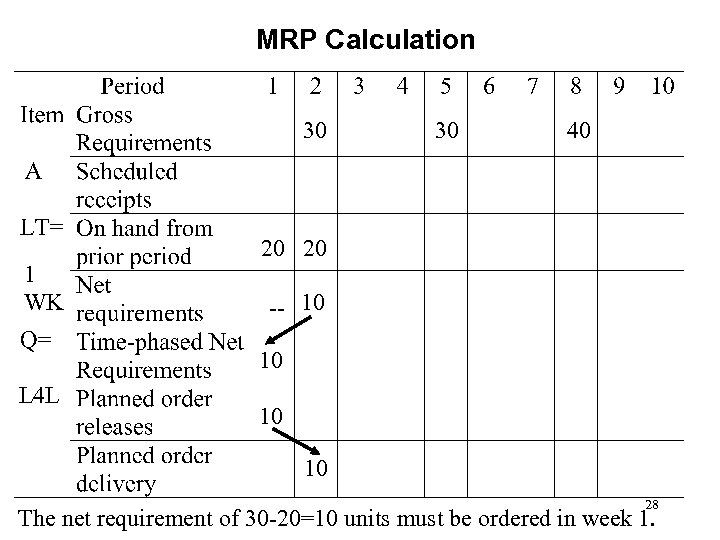MRP Calculation 30 1 WK 30 40 20 20 -- 10 10 L 4 L 10 10 28 The net requirement of 30 -20=10 units must be ordered in week 1.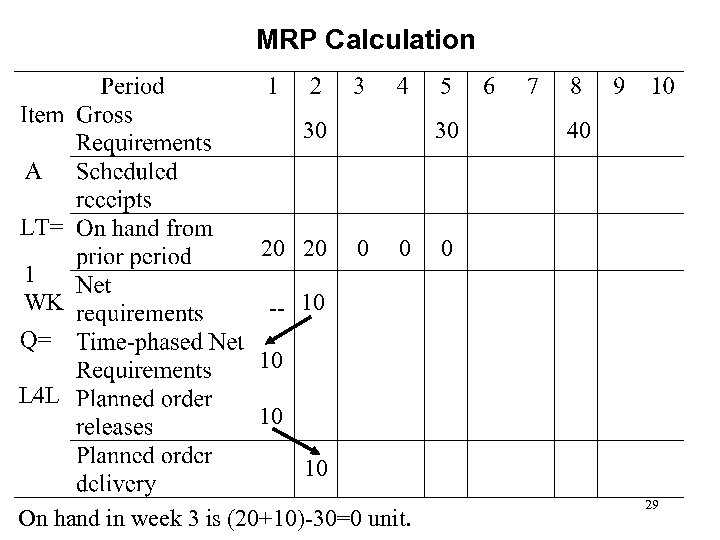MRP Calculation 30 1 WK 20 20 30 0 0 40 0 -- 10 10 L 4 L 10 10 On hand in week 3 is (20+10)-30=0 unit. 29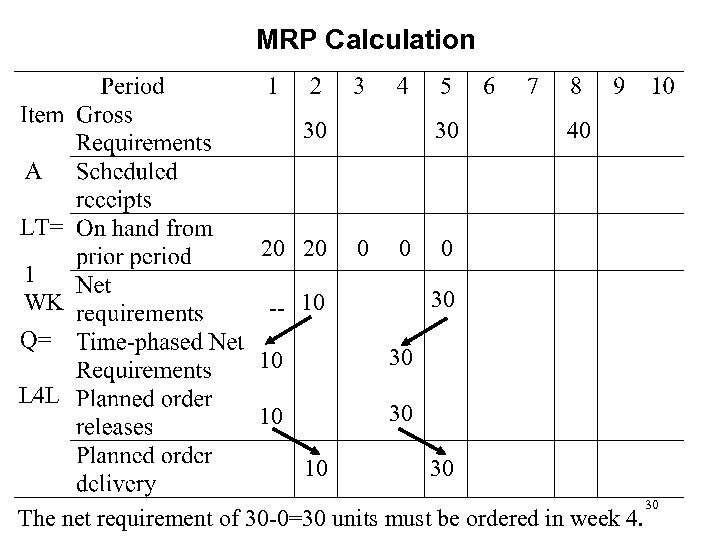MRP Calculation 30 1 WK 20 20 30 -- 10 10 L 4 L 40 30 10 30 The net requirement of 30 -0=30 units must be ordered in week 4. 30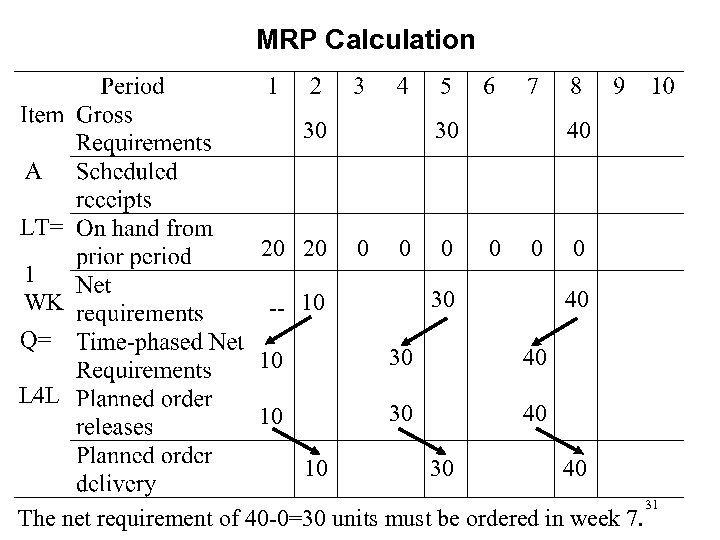MRP Calculation 30 1 WK 20 20 30 0 40 0 0 30 -- 10 40 10 L 4 L 0 30 40 10 30 40 The net requirement of 40 -0=30 units must be ordered in week 7. 31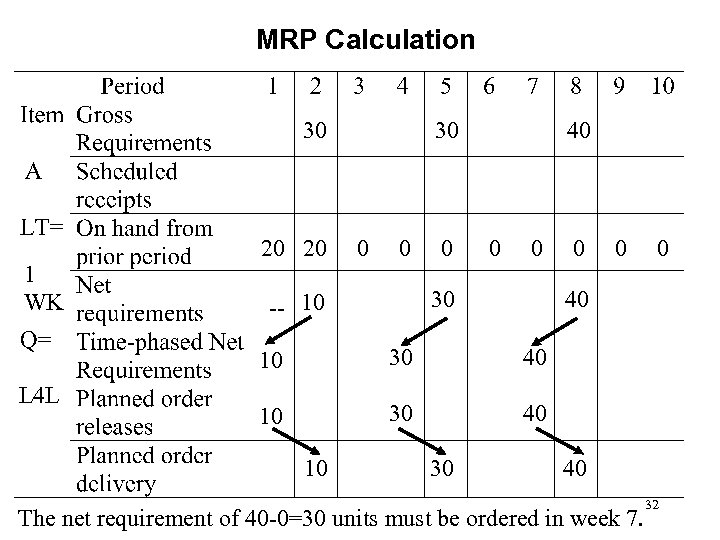MRP Calculation 30 1 WK 20 20 30 0 40 0 0 30 -- 10 0 0 40 10 L 4 L 0 30 40 10 30 40 The net requirement of 40 -0=30 units must be ordered in week 7. 32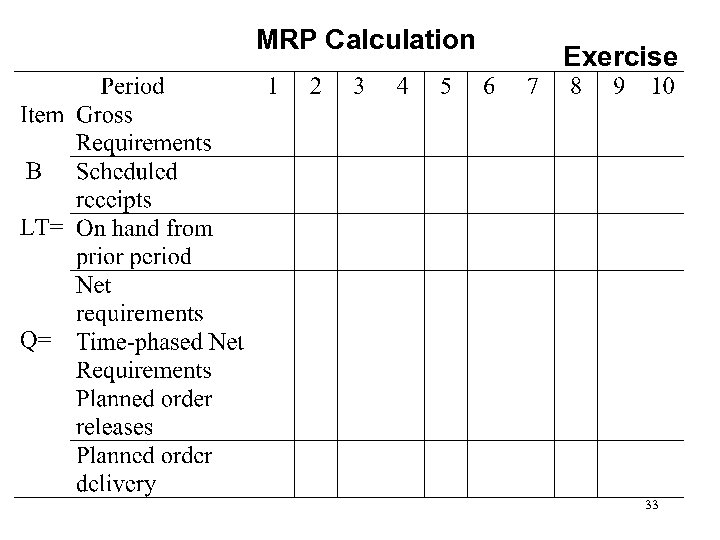MRP Calculation Exercise 33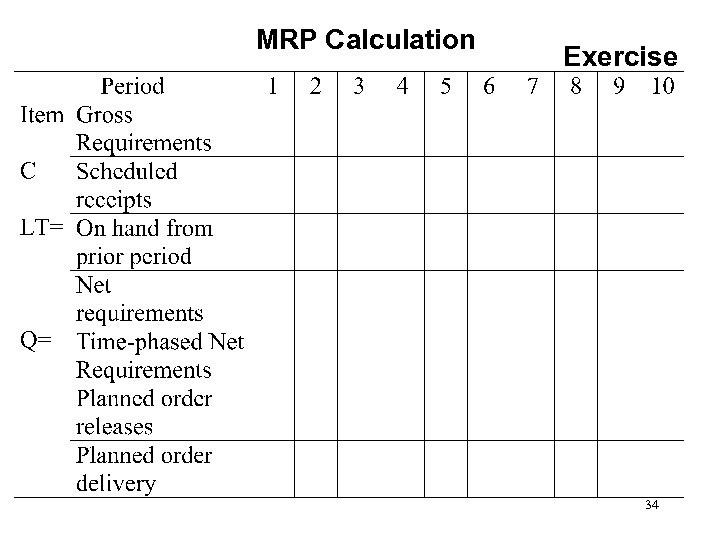MRP Calculation Exercise 34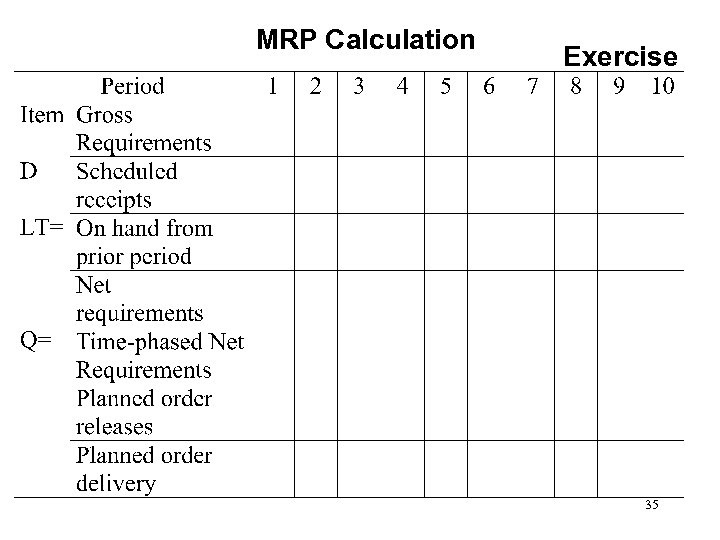MRP Calculation Exercise 35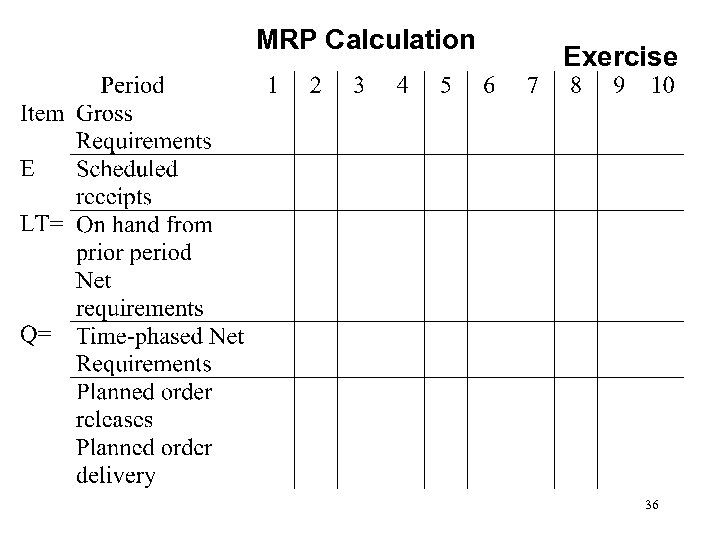MRP Calculation Exercise 36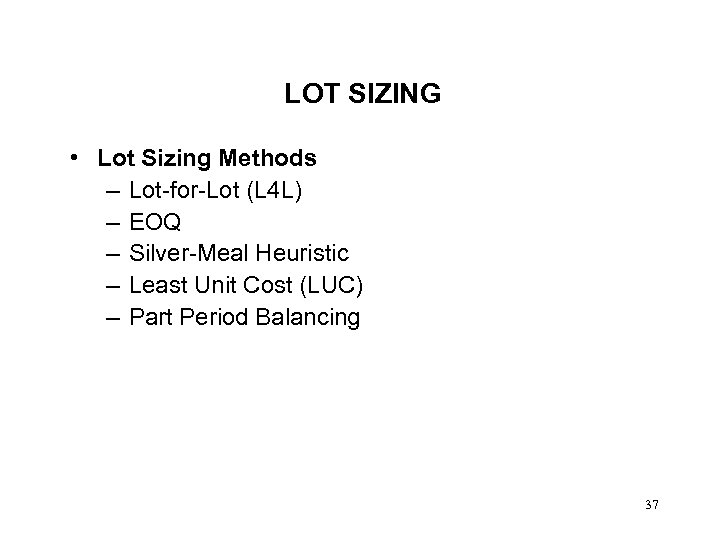LOT SIZING • Lot Sizing Methods – Lot-for-Lot (L 4 L) – EOQ – Silver-Meal Heuristic – Least Unit Cost (LUC) – Part Period Balancing 37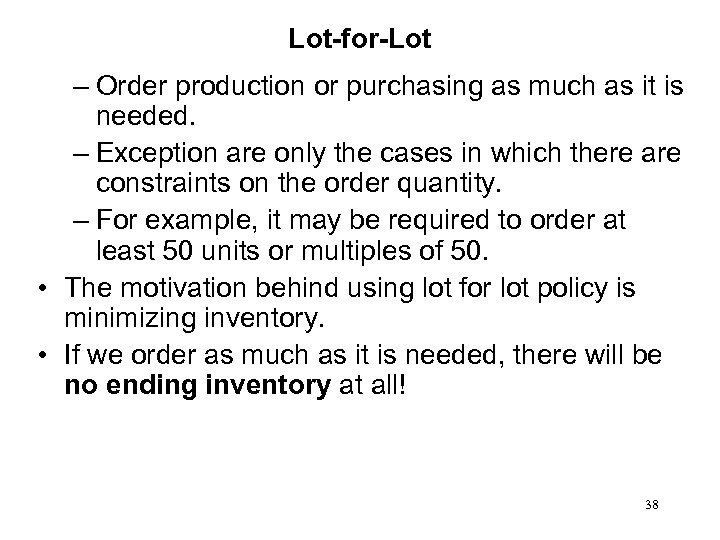Lot-for-Lot – Order production or purchasing as much as it is needed. – Exception are only the cases in which there are constraints on the order quantity. – For example, it may be required to order at least 50 units or multiples of 50. • The motivation behind using lot for lot policy is minimizing inventory. • If we order as much as it is needed, there will be no ending inventory at all! 38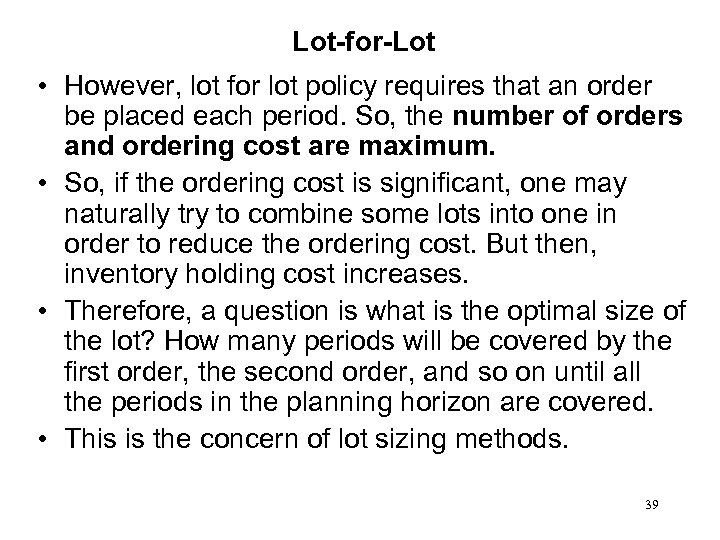Lot-for-Lot • However, lot for lot policy requires that an order be placed each period. So, the number of orders and ordering cost are maximum. • So, if the ordering cost is significant, one may naturally try to combine some lots into one in order to reduce the ordering cost. But then, inventory holding cost increases. • Therefore, a question is what is the optimal size of the lot? How many periods will be covered by the first order, the second order, and so on until all the periods in the planning horizon are covered. • This is the concern of lot sizing methods. 39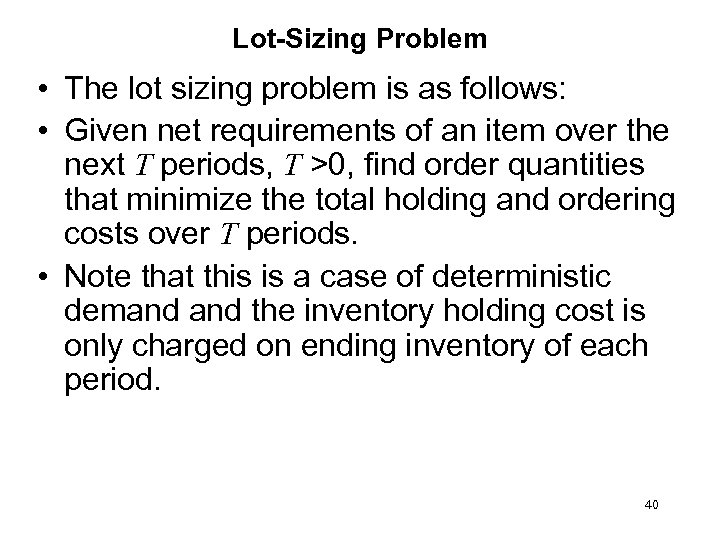Lot-Sizing Problem • The lot sizing problem is as follows: • Given net requirements of an item over the next T periods, T >0, find order quantities that minimize the total holding and ordering costs over T periods. • Note that this is a case of deterministic demand the inventory holding cost is only charged on ending inventory of each period. 40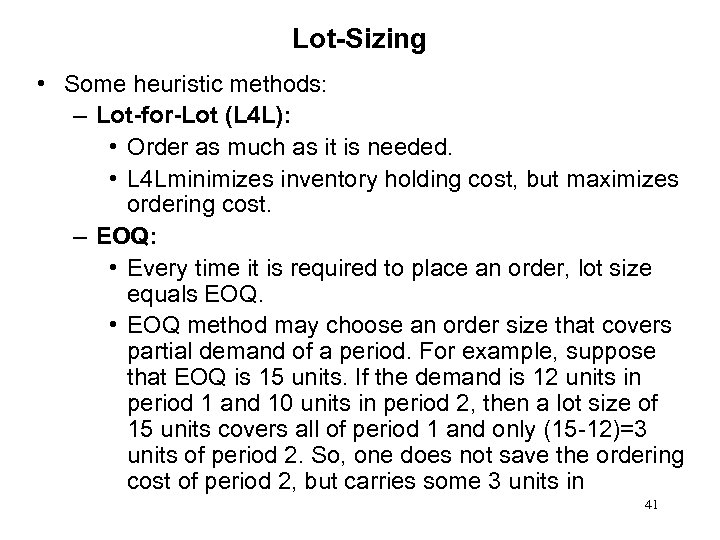Lot-Sizing • Some heuristic methods: – Lot-for-Lot (L 4 L): • Order as much as it is needed. • L 4 Lminimizes inventory holding cost, but maximizes ordering cost. – EOQ: • Every time it is required to place an order, lot size equals EOQ. • EOQ method may choose an order size that covers partial demand of a period. For example, suppose that EOQ is 15 units. If the demand is 12 units in period 1 and 10 units in period 2, then a lot size of 15 units covers all of period 1 and only (15 -12)=3 units of period 2. So, one does not save the ordering cost of period 2, but carries some 3 units in 41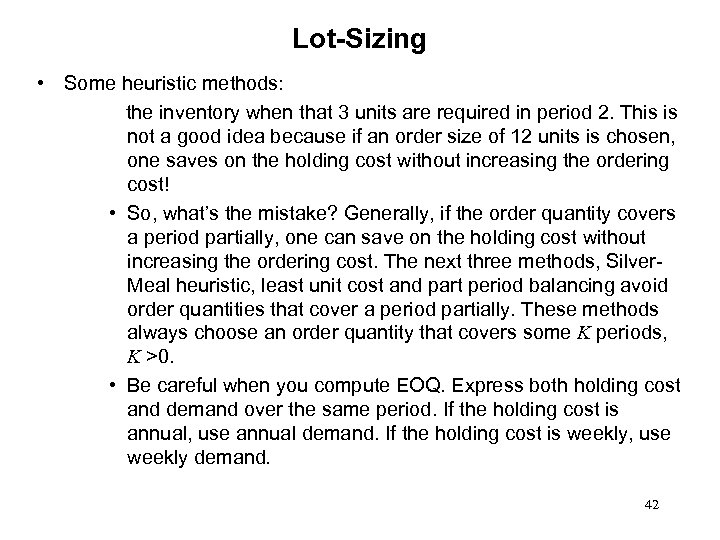Lot-Sizing • Some heuristic methods: the inventory when that 3 units are required in period 2. This is not a good idea because if an order size of 12 units is chosen, one saves on the holding cost without increasing the ordering cost! • So, what’s the mistake? Generally, if the order quantity covers a period partially, one can save on the holding cost without increasing the ordering cost. The next three methods, Silver. Meal heuristic, least unit cost and part period balancing avoid order quantities that cover a period partially. These methods always choose an order quantity that covers some K periods, K >0. • Be careful when you compute EOQ. Express both holding cost and demand over the same period. If the holding cost is annual, use annual demand. If the holding cost is weekly, use weekly demand. 42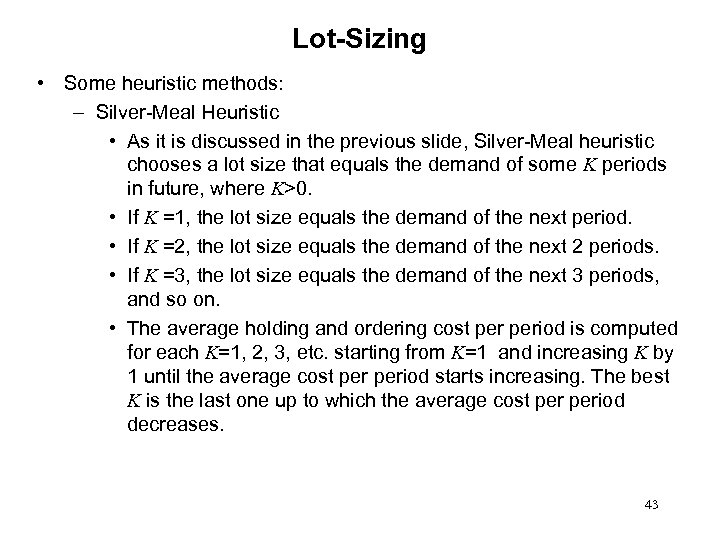Lot-Sizing • Some heuristic methods: – Silver-Meal Heuristic • As it is discussed in the previous slide, Silver-Meal heuristic chooses a lot size that equals the demand of some K periods in future, where K>0. • If K =1, the lot size equals the demand of the next period. • If K =2, the lot size equals the demand of the next 2 periods. • If K =3, the lot size equals the demand of the next 3 periods, and so on. • The average holding and ordering cost period is computed for each K=1, 2, 3, etc. starting from K=1 and increasing K by 1 until the average cost period starts increasing. The best K is the last one up to which the average cost period decreases. 43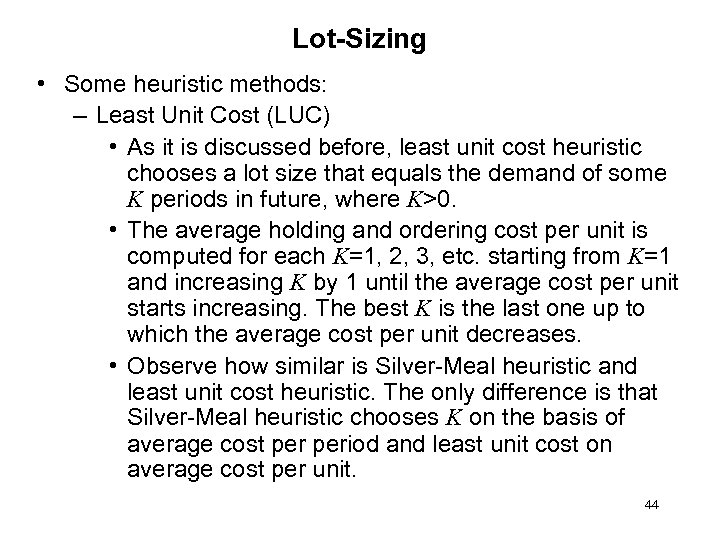Lot-Sizing • Some heuristic methods: – Least Unit Cost (LUC) • As it is discussed before, least unit cost heuristic chooses a lot size that equals the demand of some K periods in future, where K>0. • The average holding and ordering cost per unit is computed for each K=1, 2, 3, etc. starting from K=1 and increasing K by 1 until the average cost per unit starts increasing. The best K is the last one up to which the average cost per unit decreases. • Observe how similar is Silver-Meal heuristic and least unit cost heuristic. The only difference is that Silver-Meal heuristic chooses K on the basis of average cost period and least unit cost on average cost per unit. 44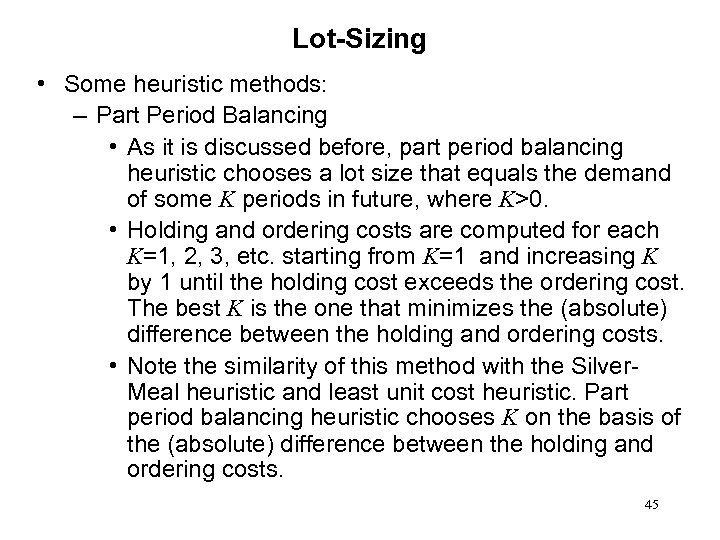Lot-Sizing • Some heuristic methods: – Part Period Balancing • As it is discussed before, part period balancing heuristic chooses a lot size that equals the demand of some K periods in future, where K>0. • Holding and ordering costs are computed for each K=1, 2, 3, etc. starting from K=1 and increasing K by 1 until the holding cost exceeds the ordering cost. The best K is the one that minimizes the (absolute) difference between the holding and ordering costs. • Note the similarity of this method with the Silver. Meal heuristic and least unit cost heuristic. Part period balancing heuristic chooses K on the basis of the (absolute) difference between the holding and ordering costs. 45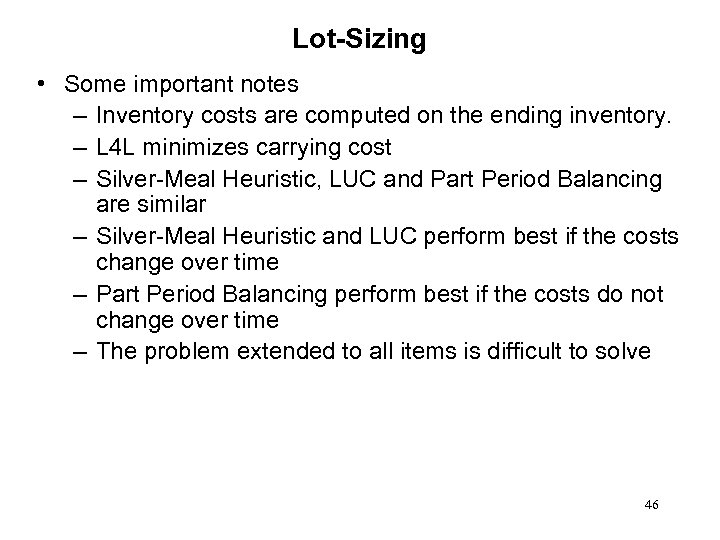Lot-Sizing • Some important notes – Inventory costs are computed on the ending inventory. – L 4 L minimizes carrying cost – Silver-Meal Heuristic, LUC and Part Period Balancing are similar – Silver-Meal Heuristic and LUC perform best if the costs change over time – Part Period Balancing perform best if the costs do not change over time – The problem extended to all items is difficult to solve 46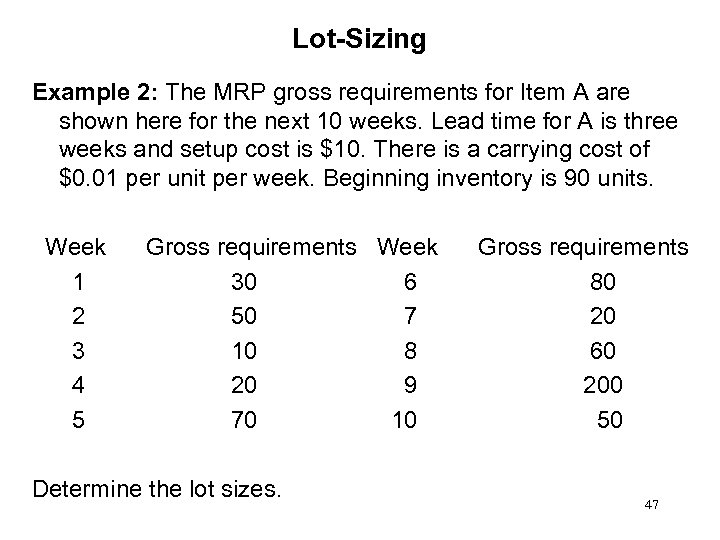Lot-Sizing Example 2: The MRP gross requirements for Item A are shown here for the next 10 weeks. Lead time for A is three weeks and setup cost is \$10. There is a carrying cost of \$0. 01 per unit per week. Beginning inventory is 90 units. Week 1 2 3 4 5 Gross requirements Week 30 6 50 7 10 8 20 9 70 10 Determine the lot sizes. Gross requirements 80 20 60 200 50 47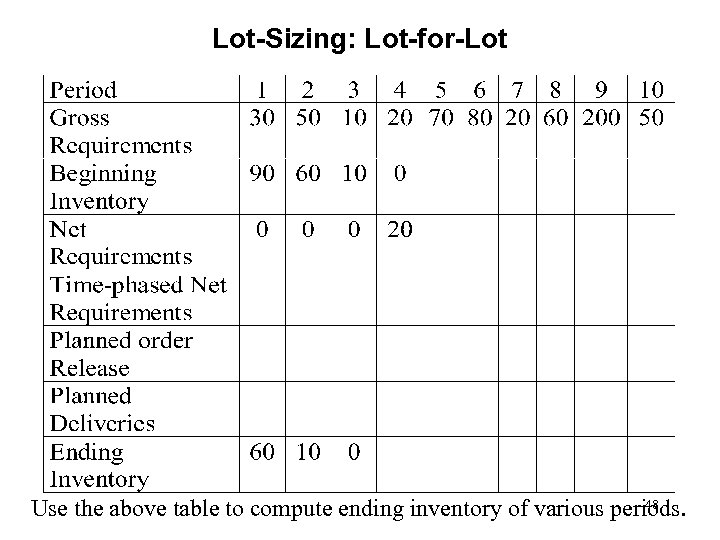Lot-Sizing: Lot-for-Lot 48 Use the above table to compute ending inventory of various periods.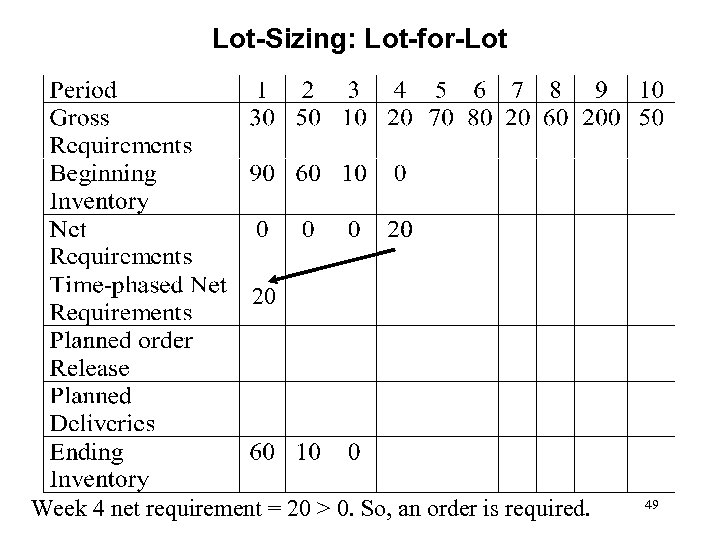Lot-Sizing: Lot-for-Lot 20 Week 4 net requirement = 20 > 0. So, an order is required. 49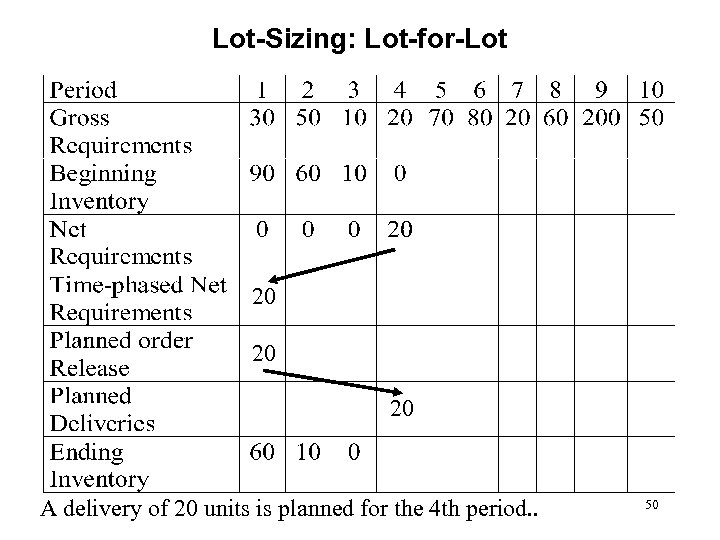Lot-Sizing: Lot-for-Lot 20 20 20 A delivery of 20 units is planned for the 4 th period. . 50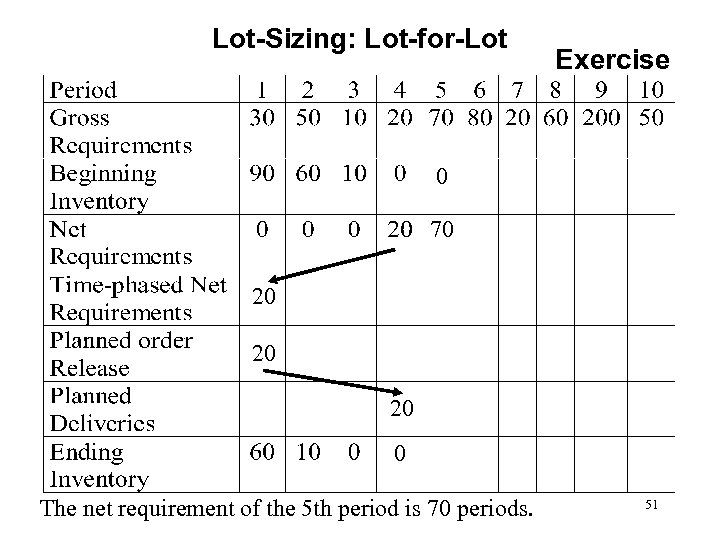Lot-Sizing: Lot-for-Lot Exercise 0 70 20 20 20 0 The net requirement of the 5 th period is 70 periods. 51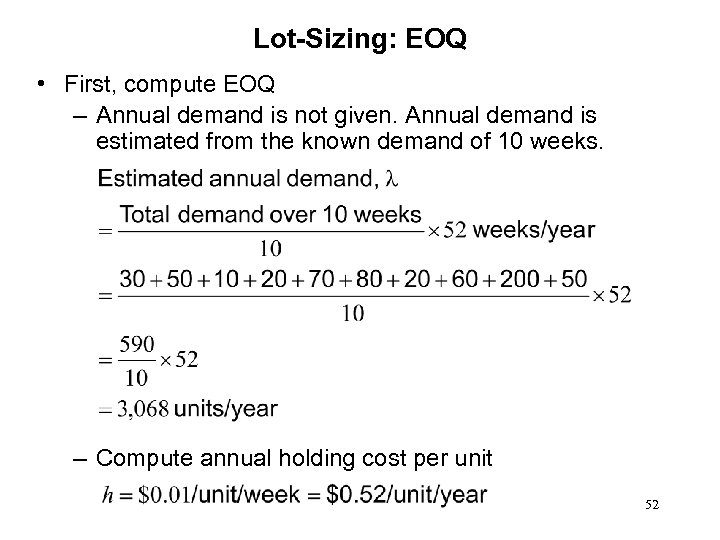Lot-Sizing: EOQ • First, compute EOQ – Annual demand is not given. Annual demand is estimated from the known demand of 10 weeks. – Compute annual holding cost per unit 52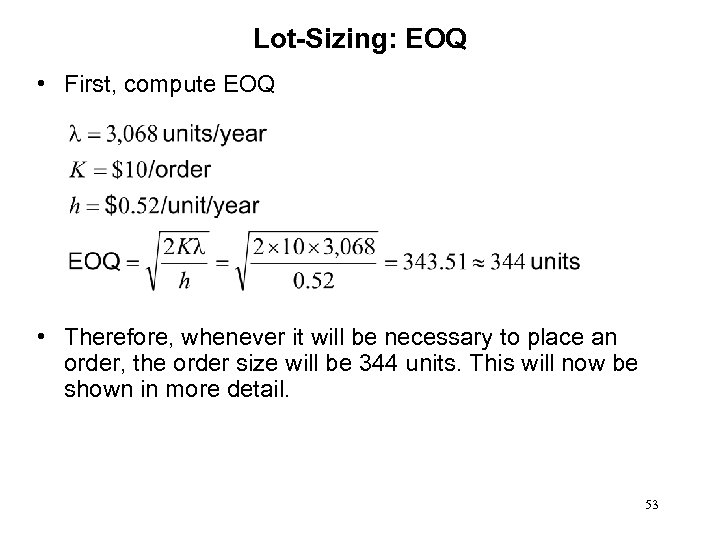Lot-Sizing: EOQ • First, compute EOQ • Therefore, whenever it will be necessary to place an order, the order size will be 344 units. This will now be shown in more detail. 53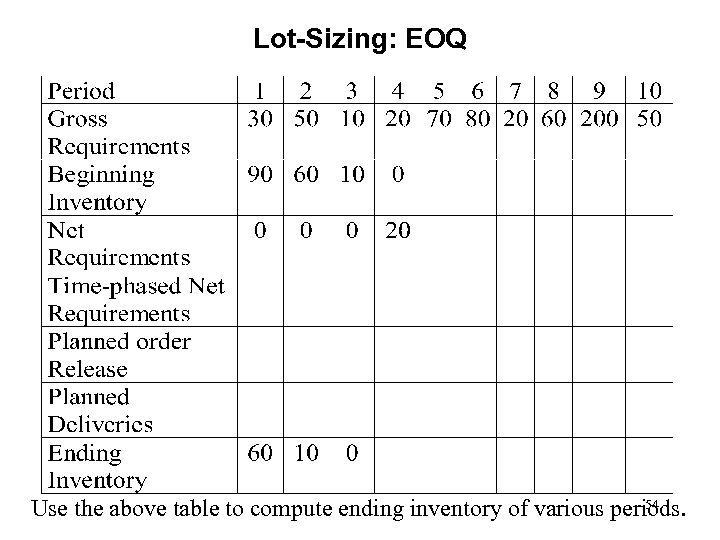Lot-Sizing: EOQ 54 Use the above table to compute ending inventory of various periods.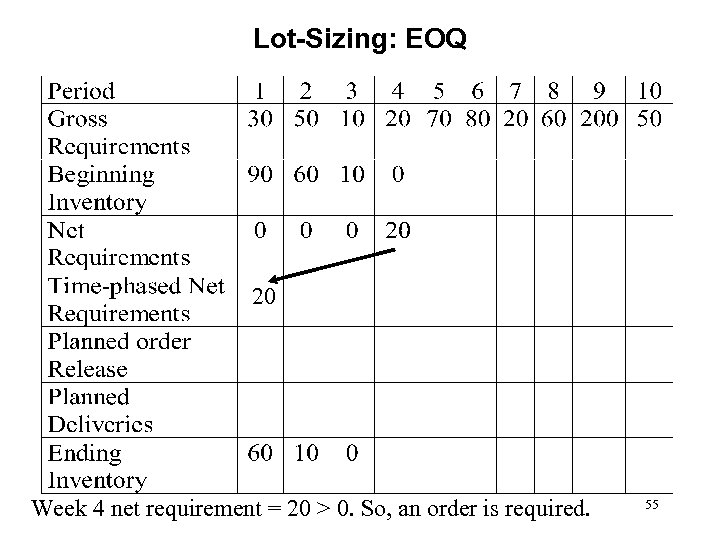Lot-Sizing: EOQ 20 Week 4 net requirement = 20 > 0. So, an order is required. 55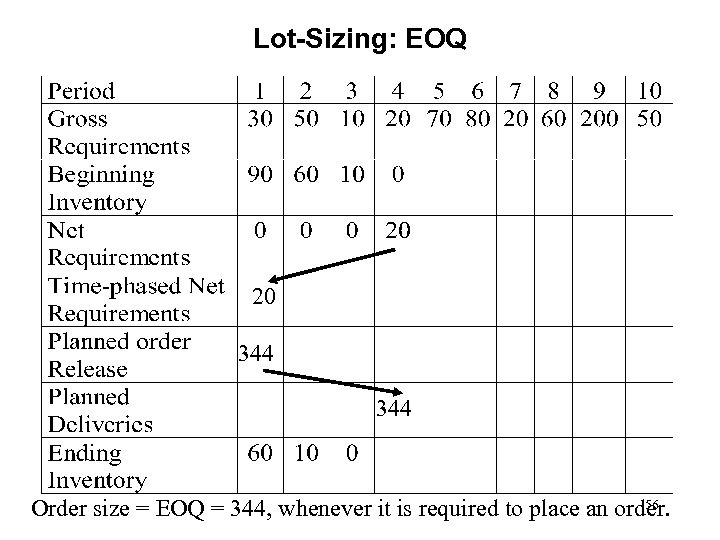Lot-Sizing: EOQ 20 344 56 Order size = EOQ = 344, whenever it is required to place an order.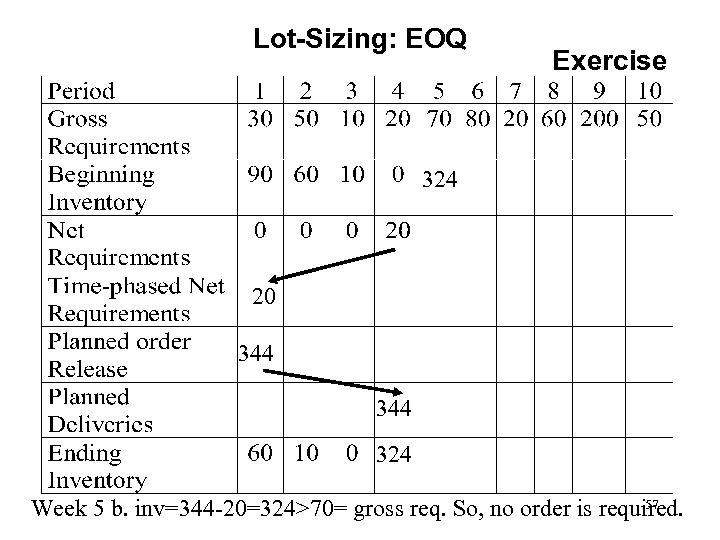Lot-Sizing: EOQ Exercise 324 20 344 324 57 Week 5 b. inv=344 -20=324>70= gross req. So, no order is required.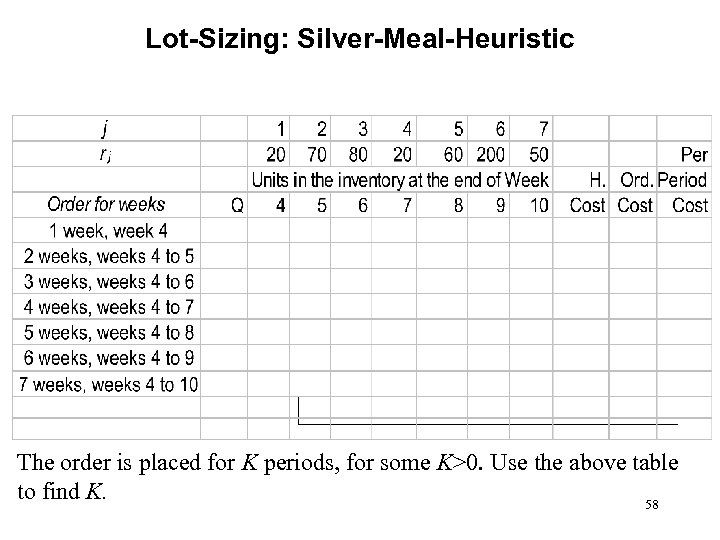Lot-Sizing: Silver-Meal-Heuristic The order is placed for K periods, for some K>0. Use the above table to find K. 58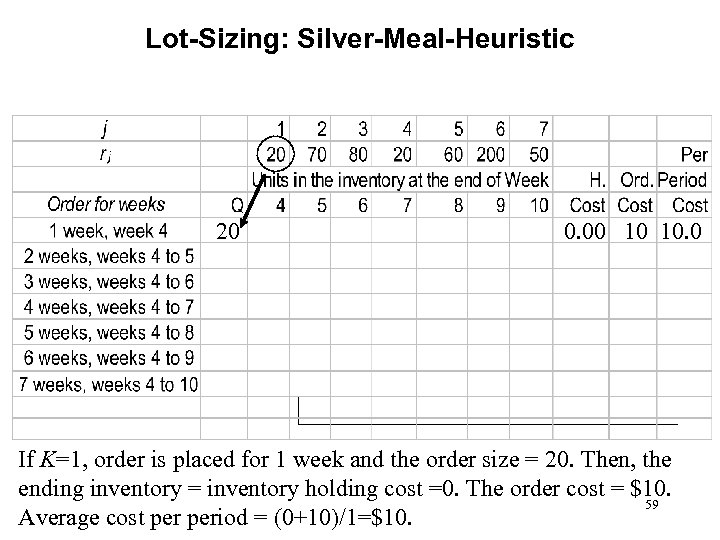Lot-Sizing: Silver-Meal-Heuristic 20 0. 00 10 10. 0 If K=1, order is placed for 1 week and the order size = 20. Then, the ending inventory = inventory holding cost =0. The order cost = \$10. 59 Average cost period = (0+10)/1=\$10.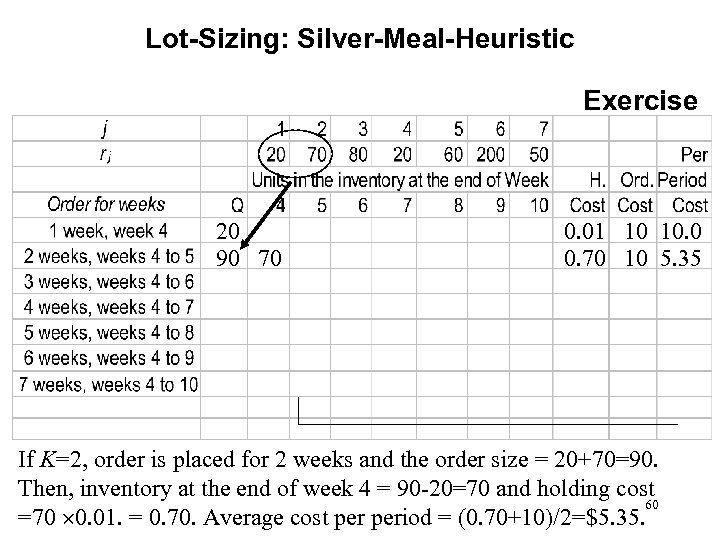Lot-Sizing: Silver-Meal-Heuristic Exercise 20 90 70 0. 01 10 10. 0 0. 70 10 5. 35 If K=2, order is placed for 2 weeks and the order size = 20+70=90. Then, inventory at the end of week 4 = 90 -20=70 and holding cost 60 =70 0. 01. = 0. 70. Average cost period = (0. 70+10)/2=\$5. 35.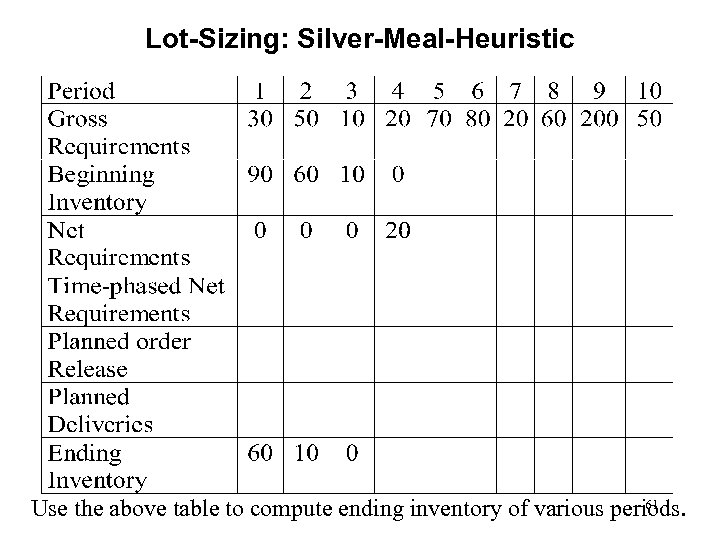Lot-Sizing: Silver-Meal-Heuristic 61 Use the above table to compute ending inventory of various periods.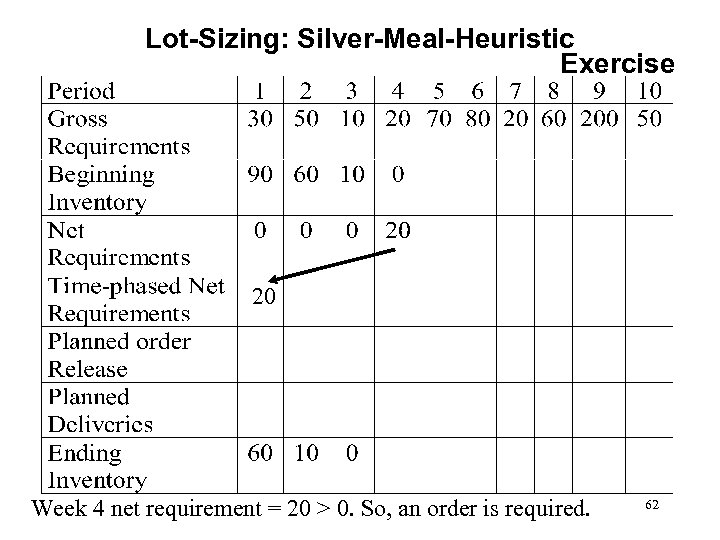Lot-Sizing: Silver-Meal-Heuristic Exercise 20 Week 4 net requirement = 20 > 0. So, an order is required. 62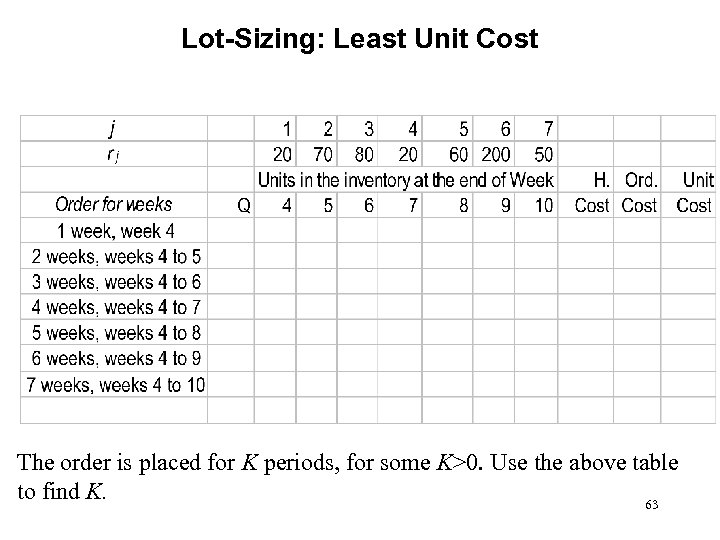Lot-Sizing: Least Unit Cost The order is placed for K periods, for some K>0. Use the above table to find K. 63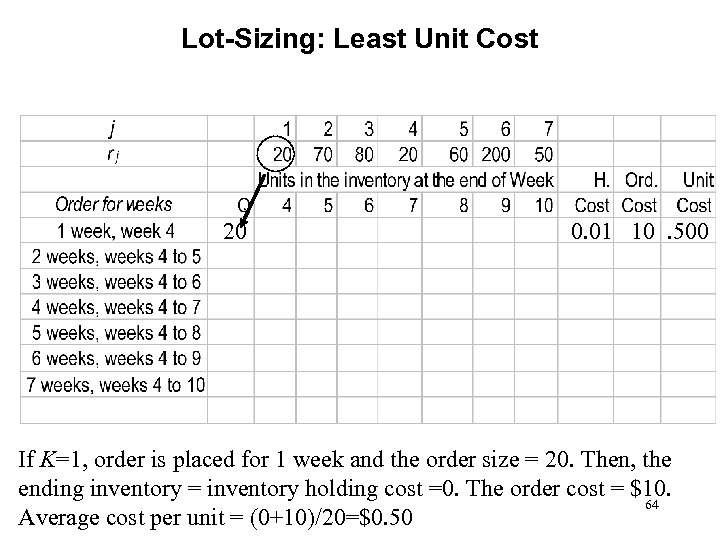Lot-Sizing: Least Unit Cost 20 0. 01 10. 500 If K=1, order is placed for 1 week and the order size = 20. Then, the ending inventory = inventory holding cost =0. The order cost = \$10. 64 Average cost per unit = (0+10)/20=\$0. 50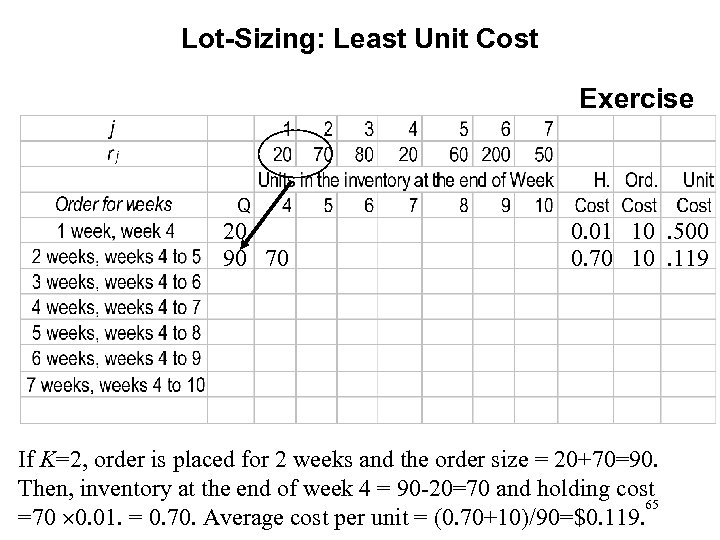Lot-Sizing: Least Unit Cost Exercise 20 90 70 0. 01 10. 500 0. 70 10. 119 If K=2, order is placed for 2 weeks and the order size = 20+70=90. Then, inventory at the end of week 4 = 90 -20=70 and holding cost 65 =70 0. 01. = 0. 70. Average cost per unit = (0. 70+10)/90=\$0. 119.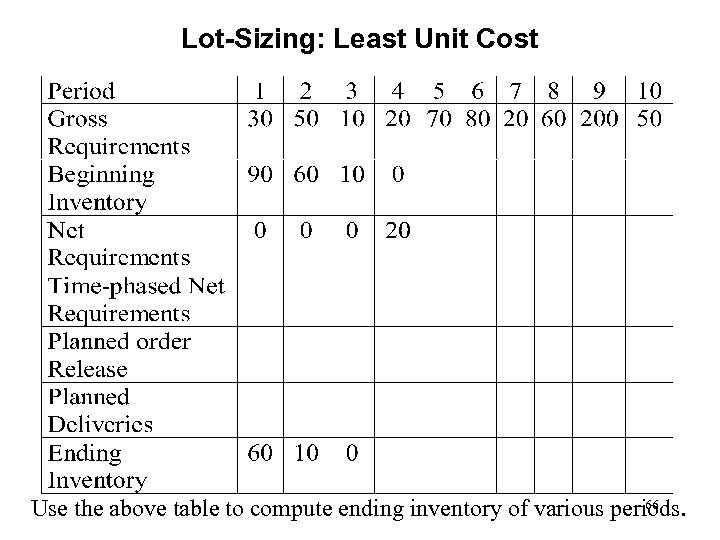Lot-Sizing: Least Unit Cost 66 Use the above table to compute ending inventory of various periods.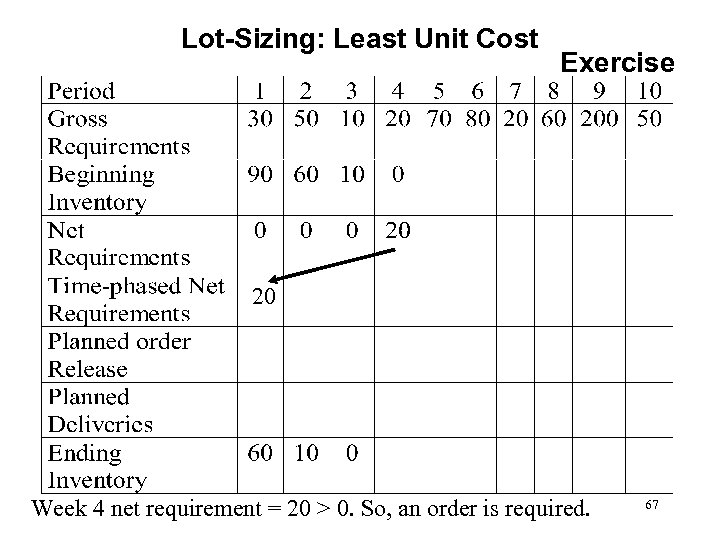Lot-Sizing: Least Unit Cost Exercise 20 Week 4 net requirement = 20 > 0. So, an order is required. 67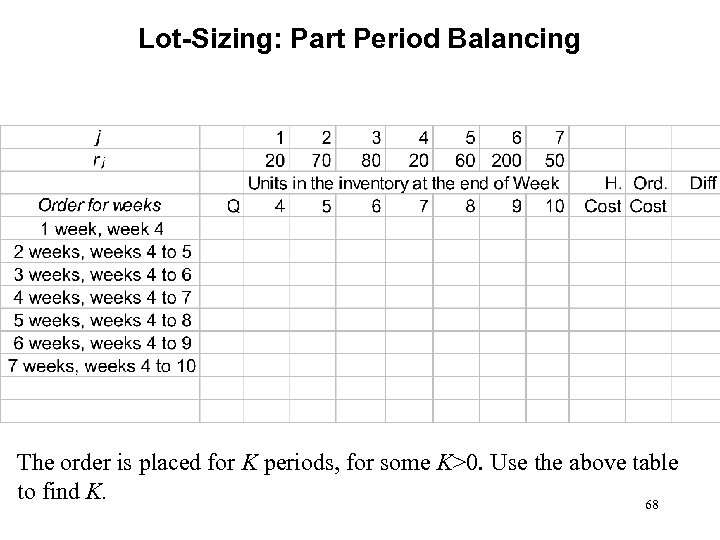Lot-Sizing: Part Period Balancing The order is placed for K periods, for some K>0. Use the above table to find K. 68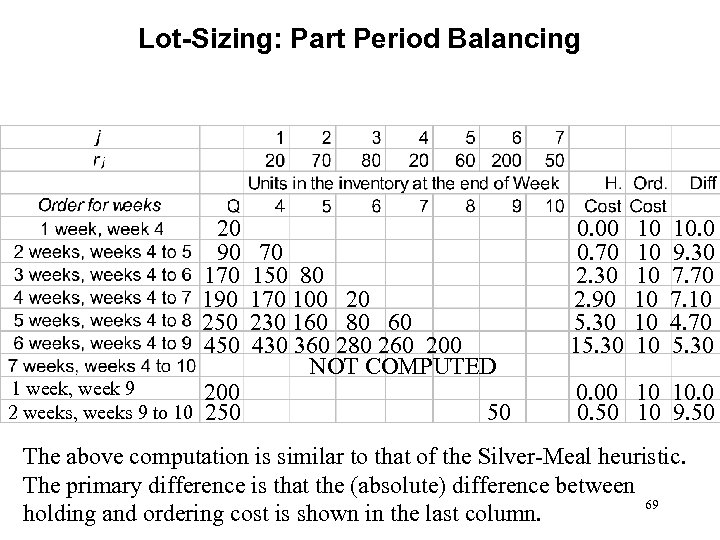Lot-Sizing: Part Period Balancing 20 90 170 190 250 450 1 week, week 9 2 weeks, weeks 9 to 10 200 250 70 150 80 170 100 20 230 160 80 60 430 360 280 260 200 NOT COMPUTED 50 0. 00 0. 70 2. 30 2. 90 5. 30 10 10. 0 9. 30 7. 70 7. 10 4. 70 5. 30 0. 00 10 10. 0 0. 50 10 9. 50 The above computation is similar to that of the Silver-Meal heuristic. The primary difference is that the (absolute) difference between 69 holding and ordering cost is shown in the last column.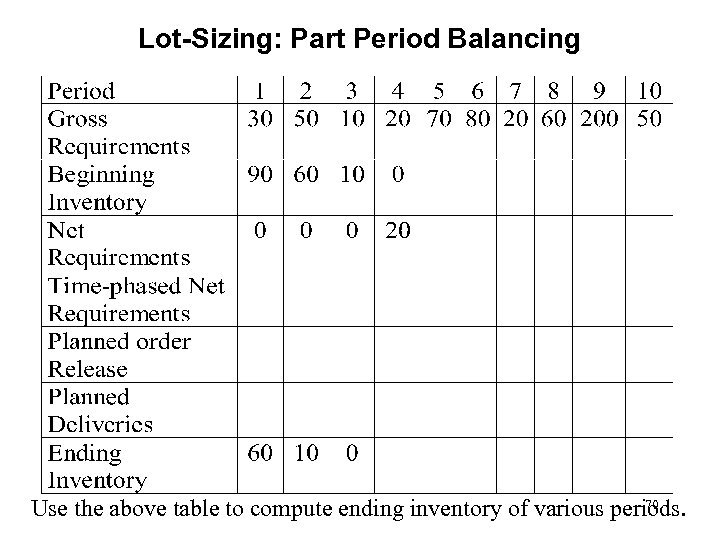Lot-Sizing: Part Period Balancing 70 Use the above table to compute ending inventory of various periods.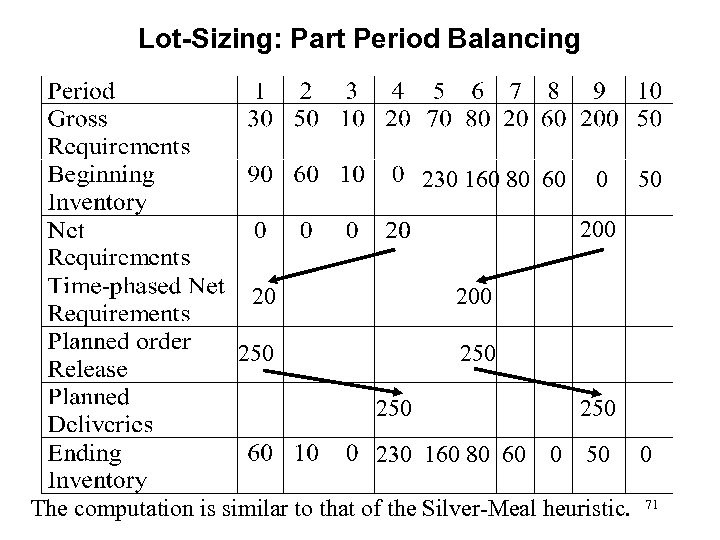Lot-Sizing: Part Period Balancing 230 160 80 60 0 50 200 250 250 230 160 80 60 250 0 50 The computation is similar to that of the Silver-Meal heuristic. 0 71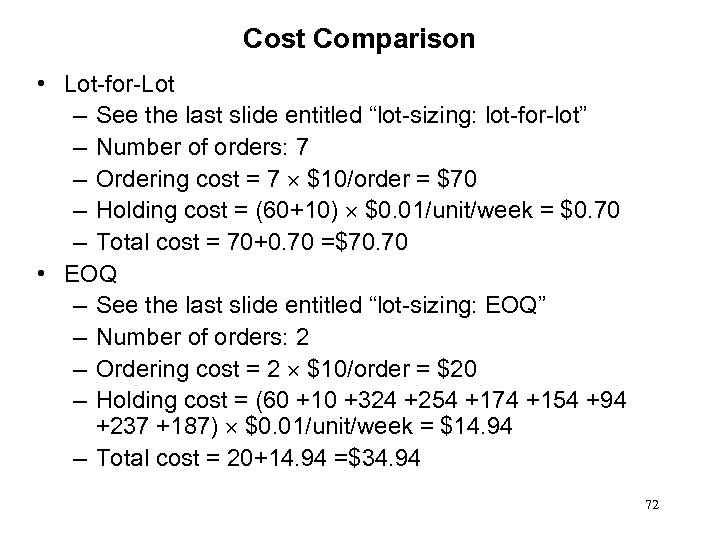Cost Comparison • Lot-for-Lot – See the last slide entitled “lot-sizing: lot-for-lot” – Number of orders: 7 – Ordering cost = 7 \$10/order = \$70 – Holding cost = (60+10) \$0. 01/unit/week = \$0. 70 – Total cost = 70+0. 70 =\$70. 70 • EOQ – See the last slide entitled “lot-sizing: EOQ” – Number of orders: 2 – Ordering cost = 2 \$10/order = \$20 – Holding cost = (60 +10 +324 +254 +174 +154 +94 +237 +187) \$0. 01/unit/week = \$14. 94 – Total cost = 20+14. 94 =\$34. 94 72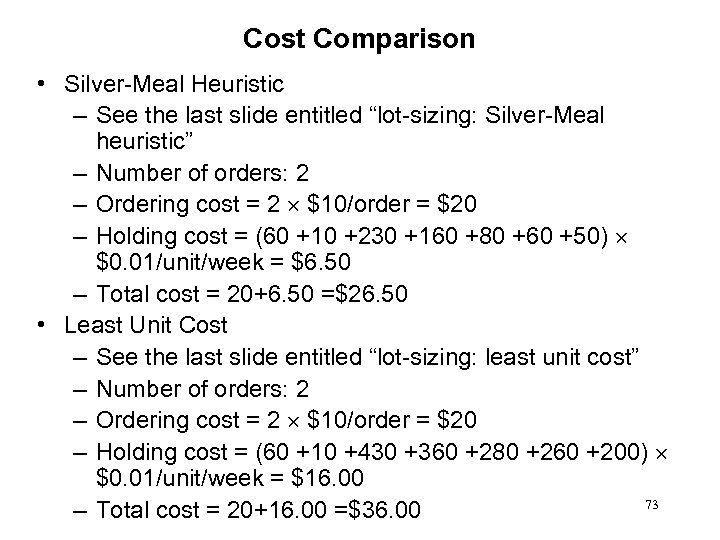Cost Comparison • Silver-Meal Heuristic – See the last slide entitled “lot-sizing: Silver-Meal heuristic” – Number of orders: 2 – Ordering cost = 2 \$10/order = \$20 – Holding cost = (60 +10 +230 +160 +80 +60 +50) \$0. 01/unit/week = \$6. 50 – Total cost = 20+6. 50 =\$26. 50 • Least Unit Cost – See the last slide entitled “lot-sizing: least unit cost” – Number of orders: 2 – Ordering cost = 2 \$10/order = \$20 – Holding cost = (60 +10 +430 +360 +280 +260 +200) \$0. 01/unit/week = \$16. 00 73 – Total cost = 20+16. 00 =\$36. 00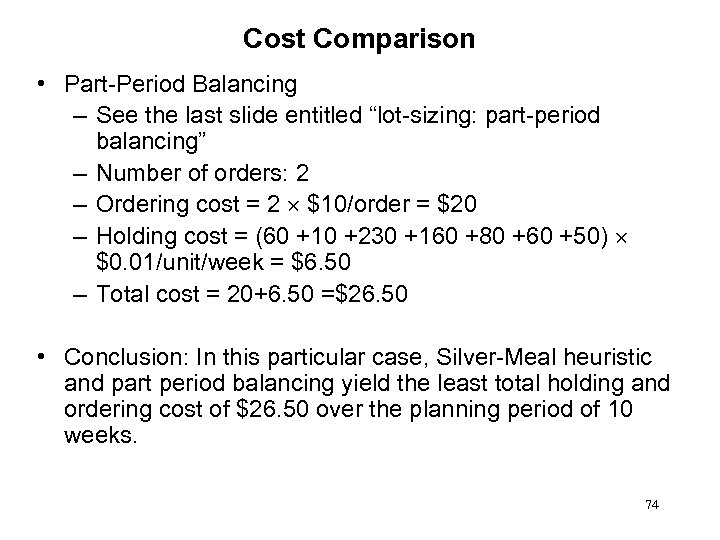Cost Comparison • Part-Period Balancing – See the last slide entitled “lot-sizing: part-period balancing” – Number of orders: 2 – Ordering cost = 2 \$10/order = \$20 – Holding cost = (60 +10 +230 +160 +80 +60 +50) \$0. 01/unit/week = \$6. 50 – Total cost = 20+6. 50 =\$26. 50 • Conclusion: In this particular case, Silver-Meal heuristic and part period balancing yield the least total holding and ordering cost of \$26. 50 over the planning period of 10 weeks. 74